## RATIO

1 ) Write down the condition for which x : y becomes ratio of less inequality.

Solution : x < y

2 ) Under which condition x : y becomes ratio of greater inequality ?

Solution : x > y

3 ) Under which condition x : y becomes ratio of greater equality ?

Solution : x = y

4 ) Write down the inverse ratio of pr : qr in its lowest term.

Solution :

The required inverse ratio = qr : pr = q : p

5 ) Write down the inverse ratio of x yp : xyp in its lowest term.

Solution :

Required inverse ratio is y : x

6 ) If the ratio of lowest term of two positive real numbers is p : q and their HCF is r, what are the two positive numbers ?

7 ) If the ratio of two positive numbers is 2 : 3 and their HCF is 7, find out the numbers.

Solution :

Ratio = 2 : 3

HCF = 7

First number = 2 * 7 = 14

Second number = 3 * 7 = 21

8 ) Find out the mixed ratio of a : b, p : q, x : y.

Solution :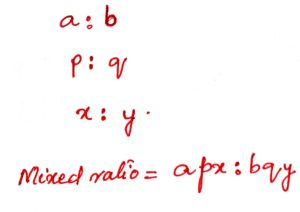9 ) Find out the compound ratio of the following ratios :

a : bc, b : ca, c : ab.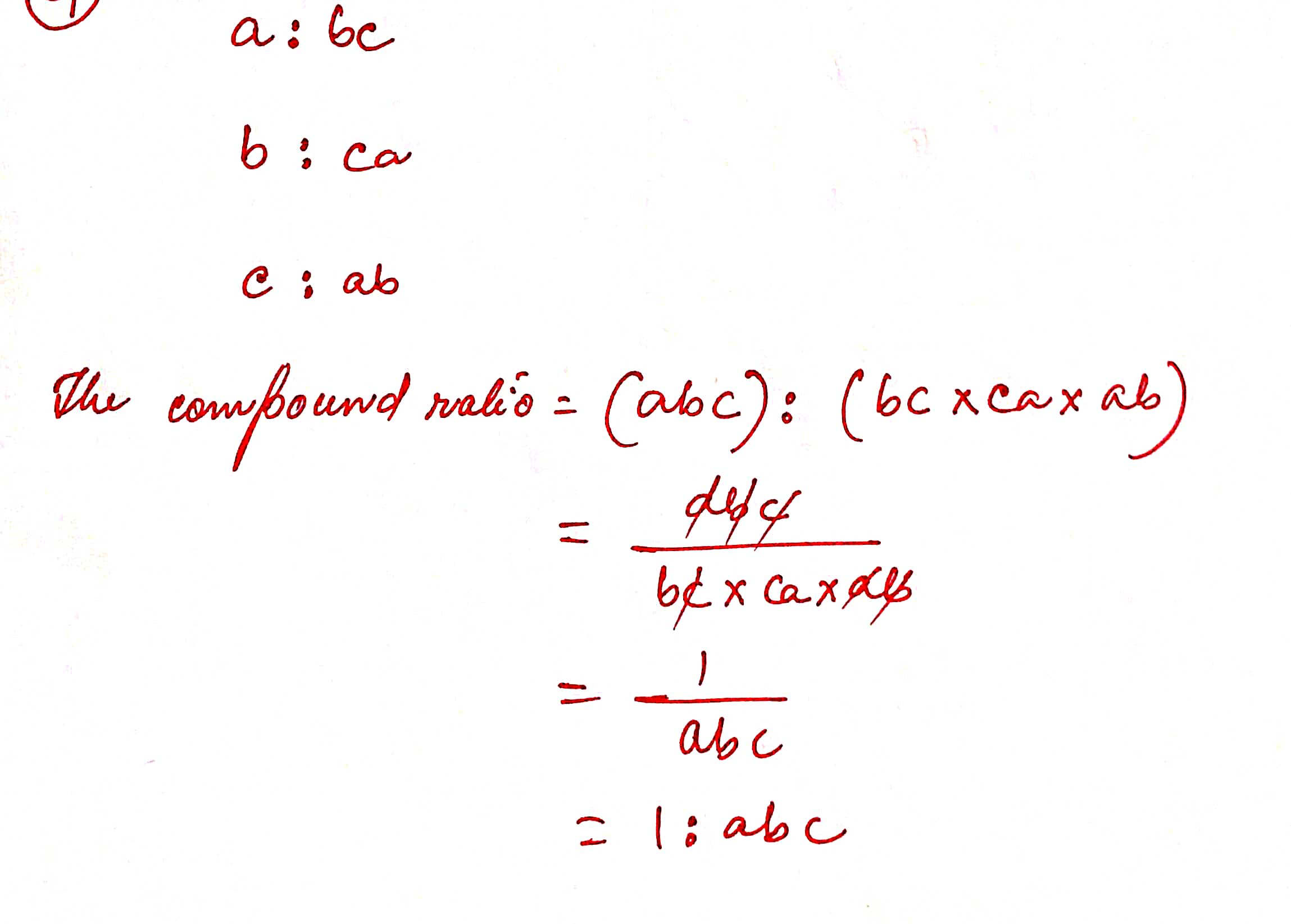10 ) What is the compound ratio of the ratios pq : r and r : pq ?

Solution :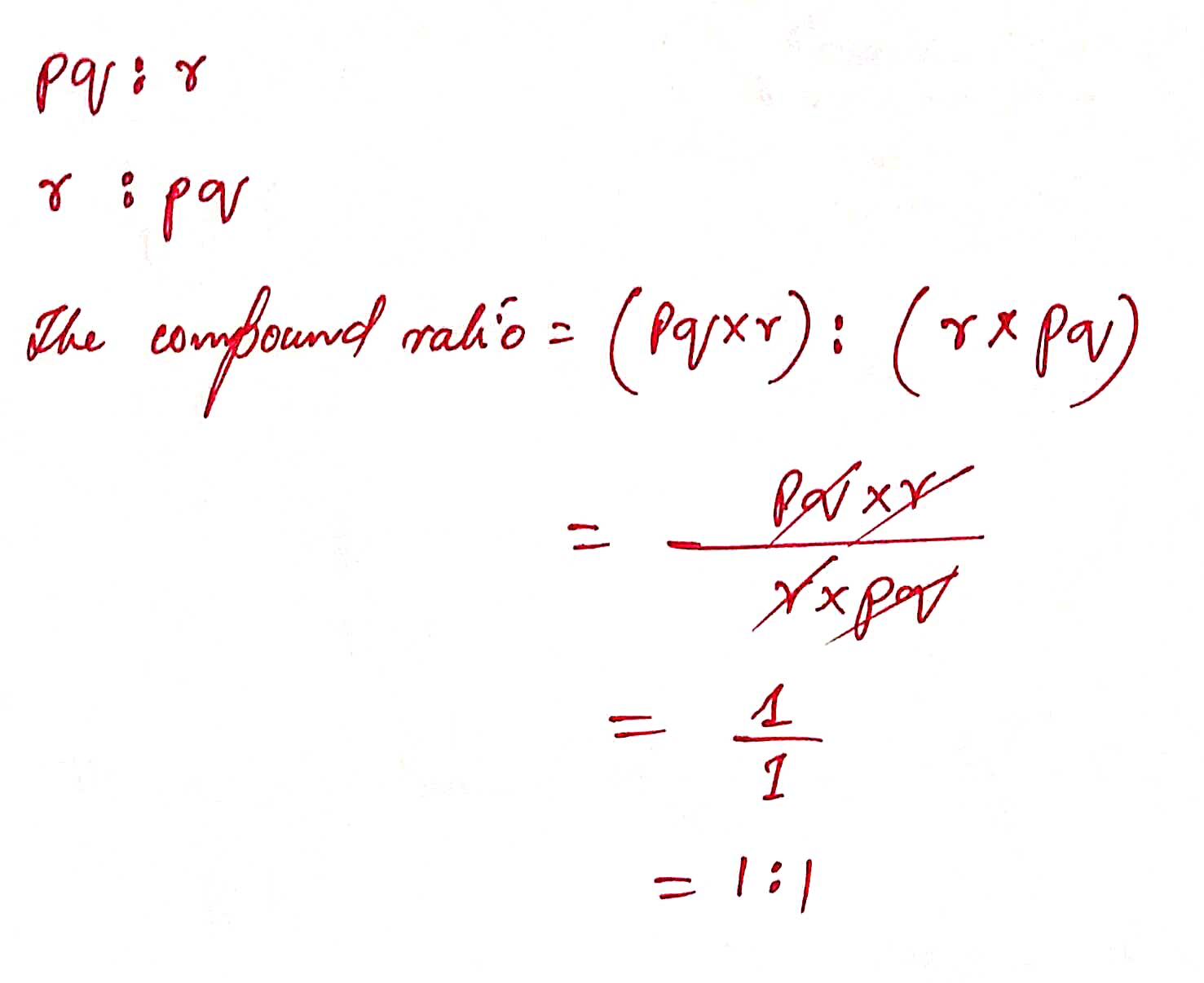11 ) Find out the mixed ratio of a/b : c , b/c : a , c/a : b .

Solution :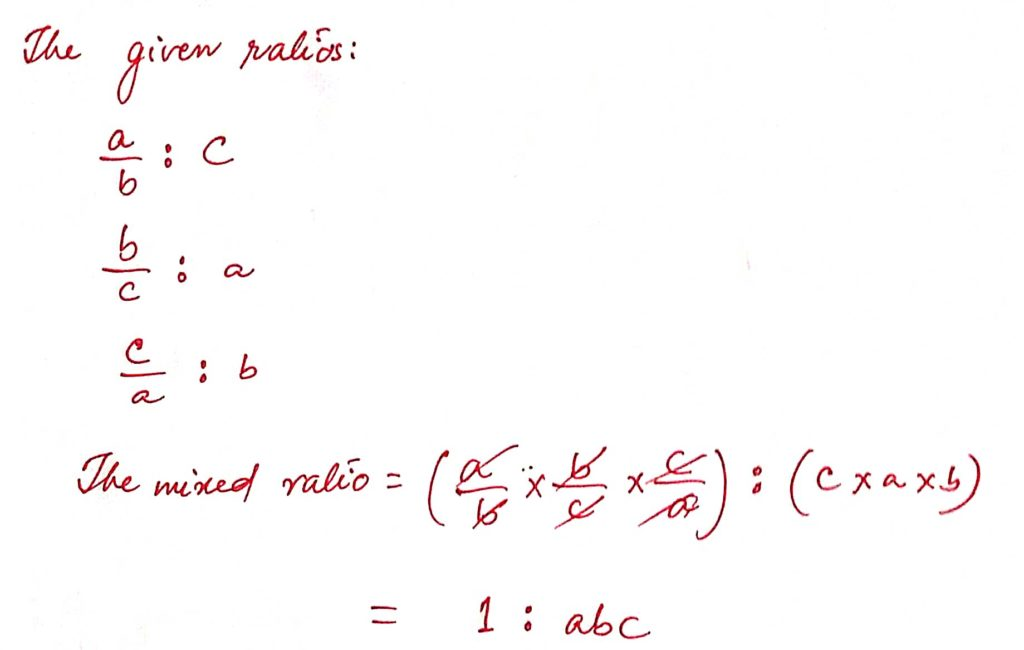12 ) Find the mixed ratio of the inverse ratio a/b :c, b/c : a, c/a : b

Solution :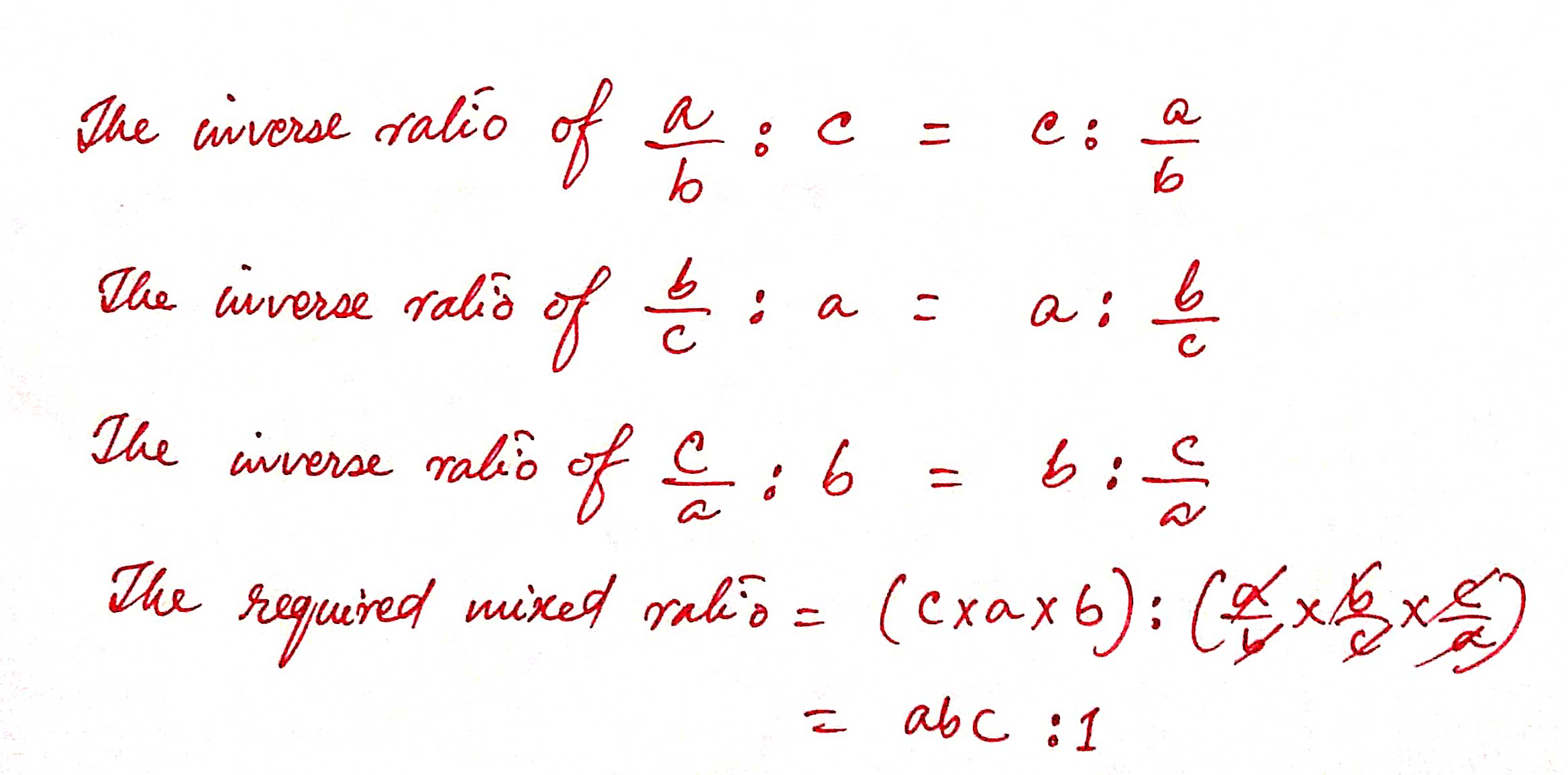13 ) What will be the compound ratio of the inverse ratio of the ratios : x : yz/x, y : zx/y and z : yx/z ?

Solution :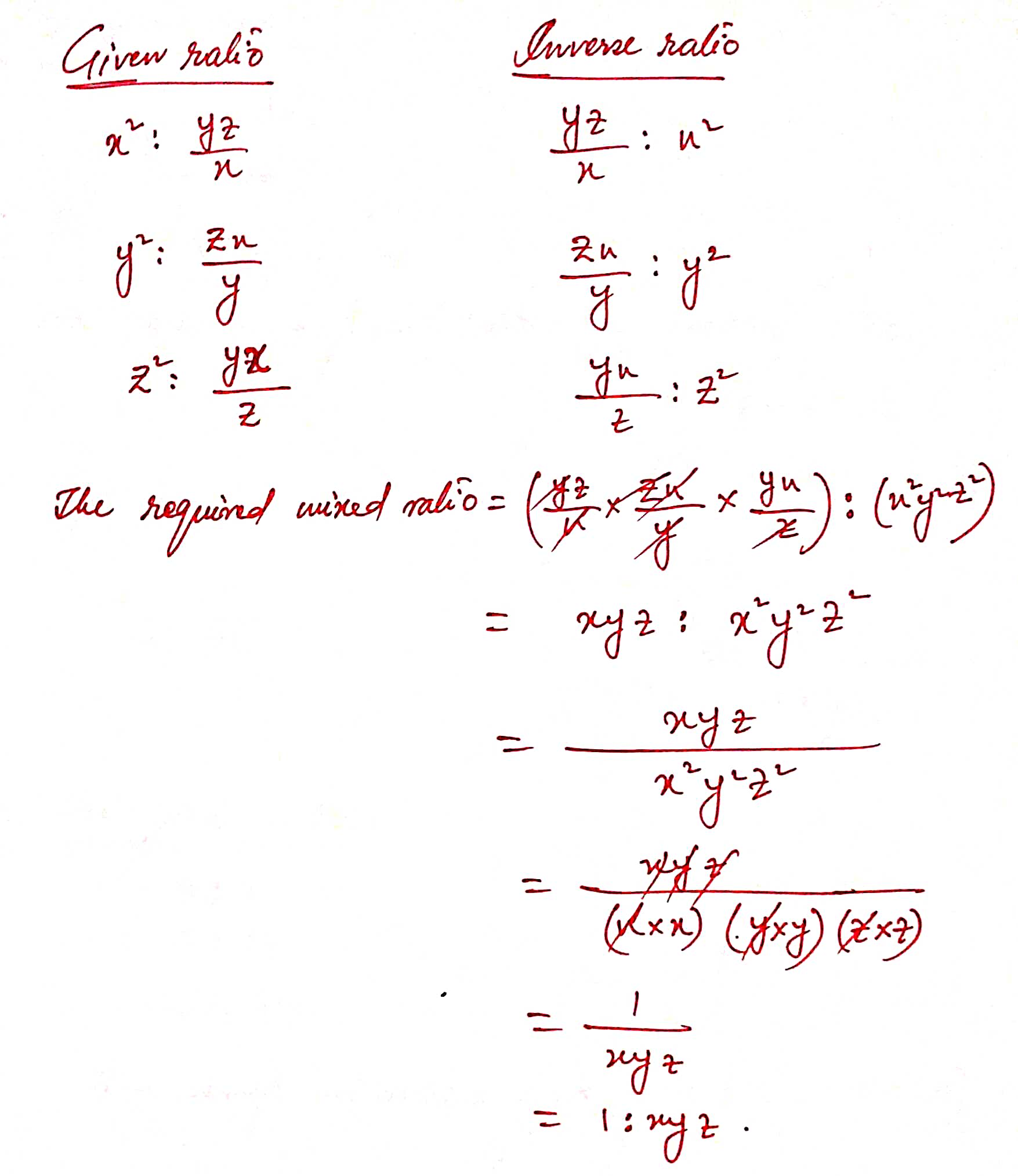14 ) Find the mixed ratio of the ratios 4 : 5, 5 : 7 and 9 : 11.

Solution :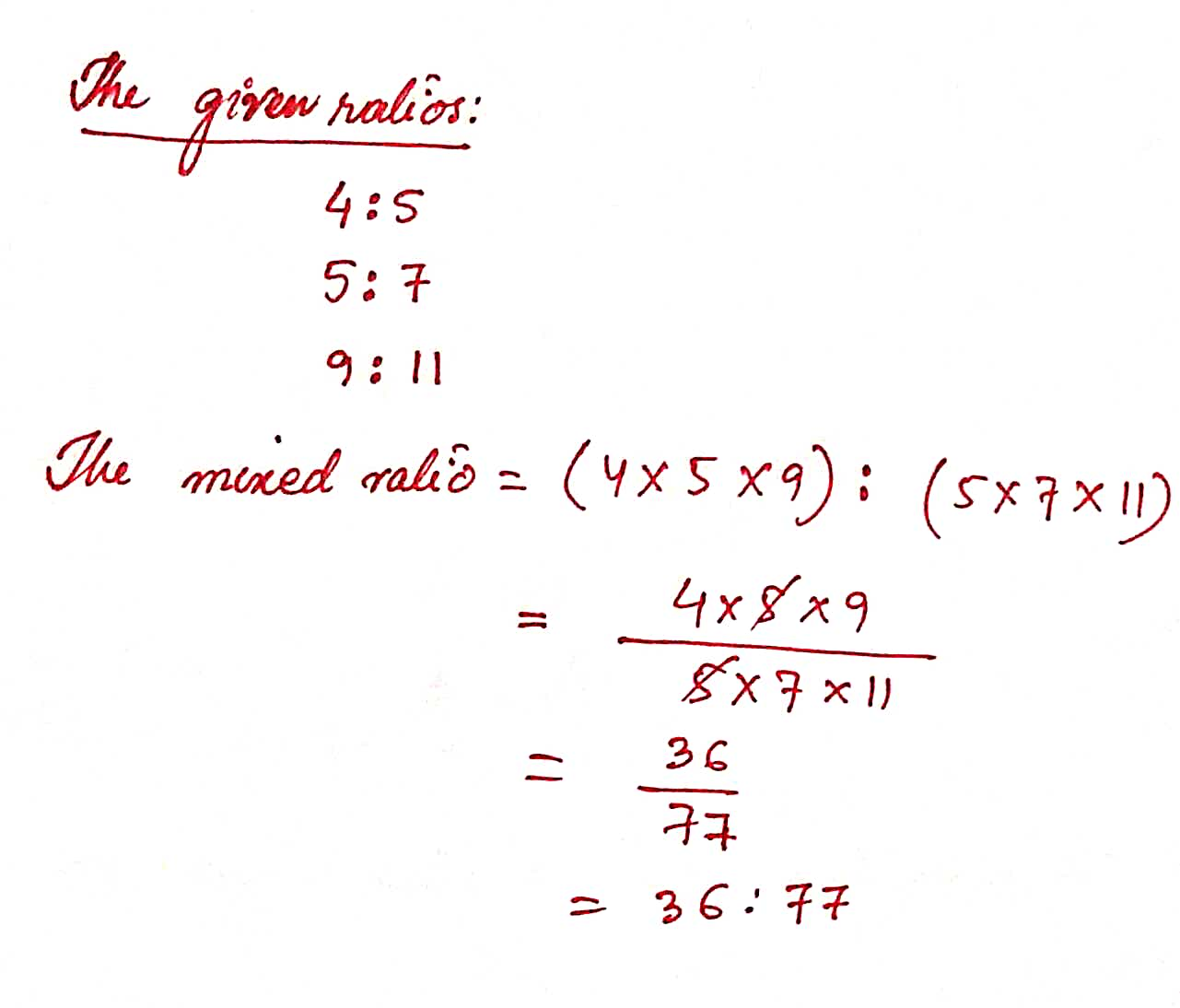15 ) Find the compound ratio of the ratios 3a : 2b, 2m : n , 4x : 3y.

Solution :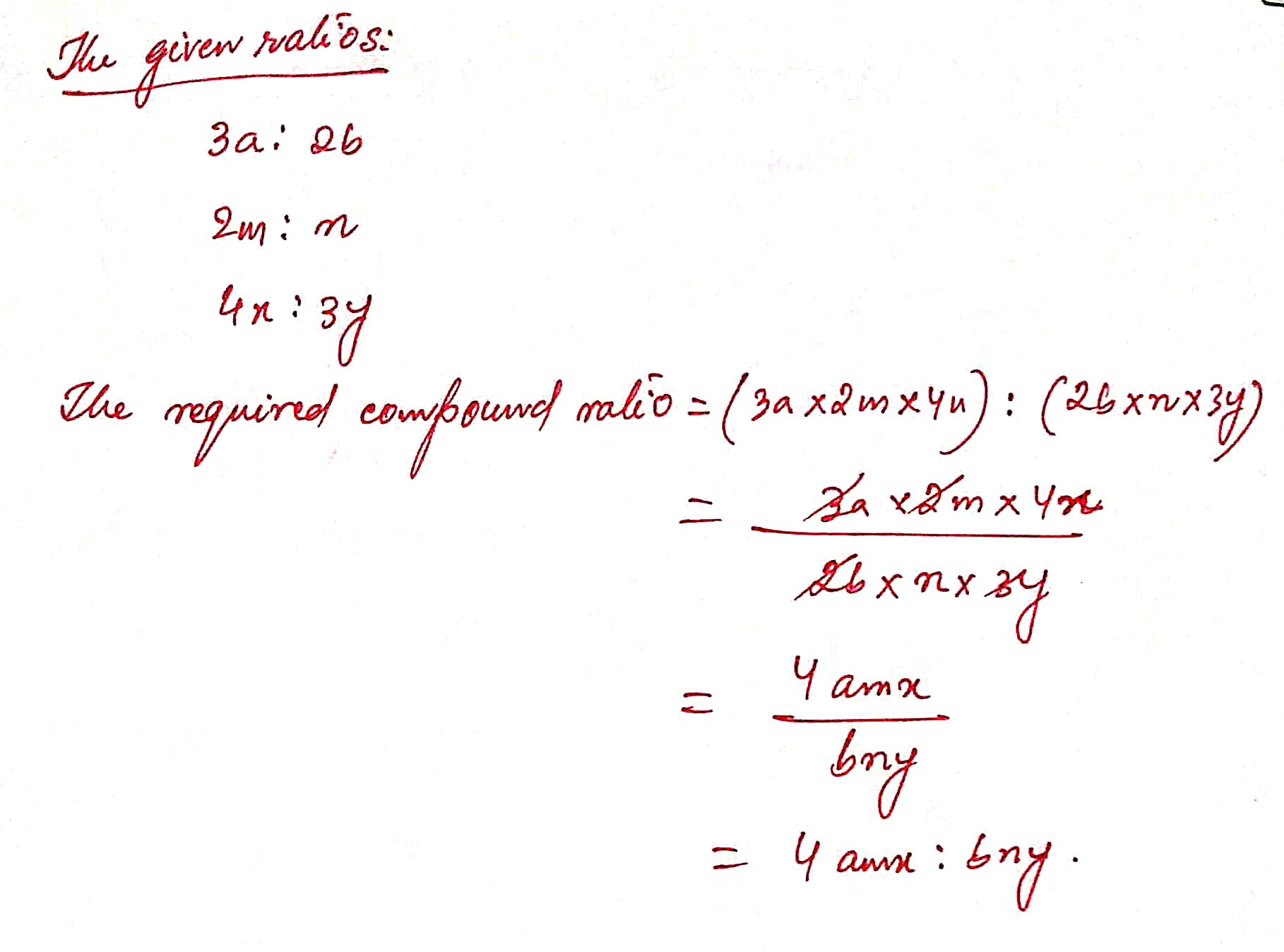16 ) What will be the compound ratio of 2 : 3, 9 : 14 and 14 : 27 ?

Solution :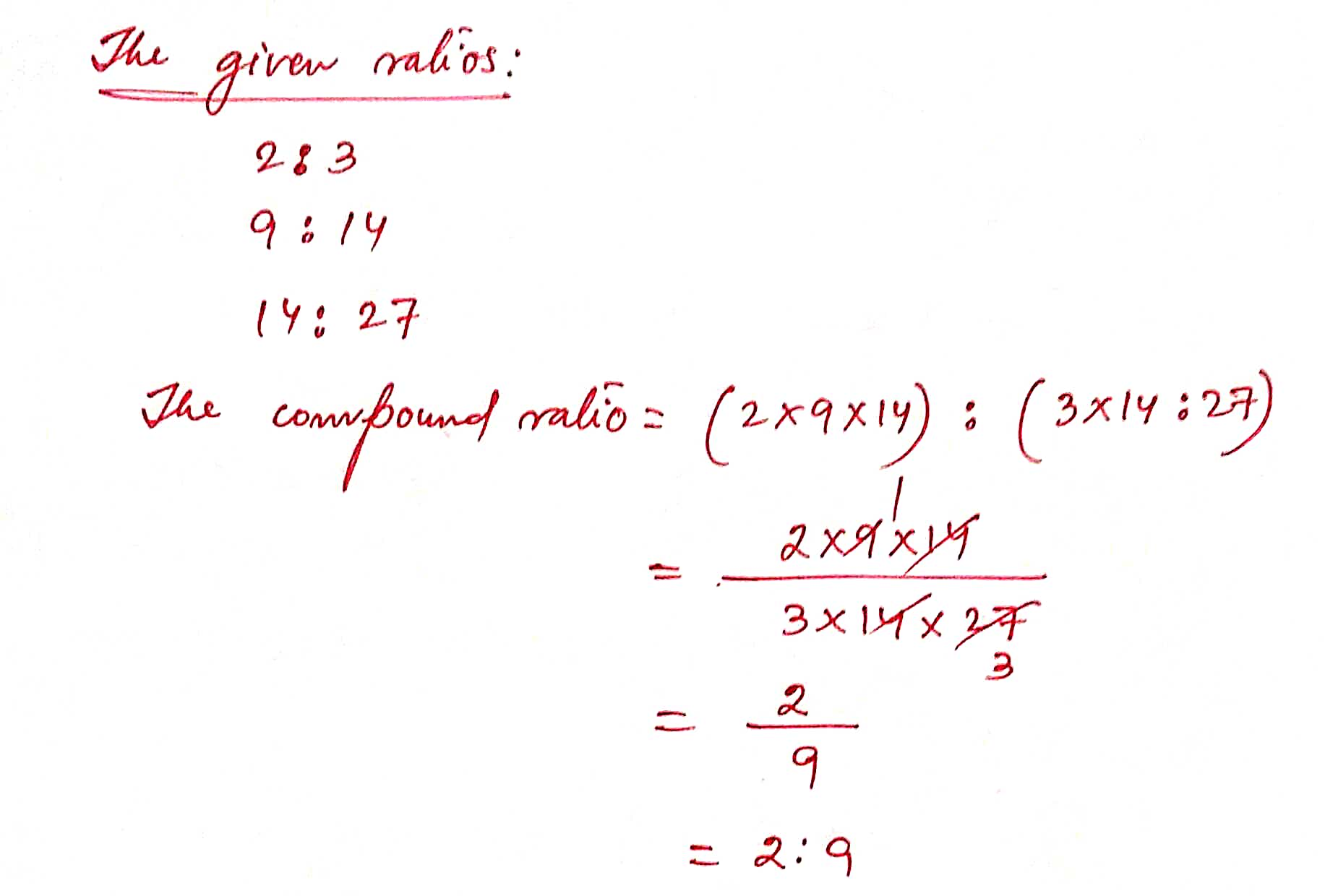17 ) Find the mixed ratio of 2 : 1, 3 : 5, 20 : 9.

Solution :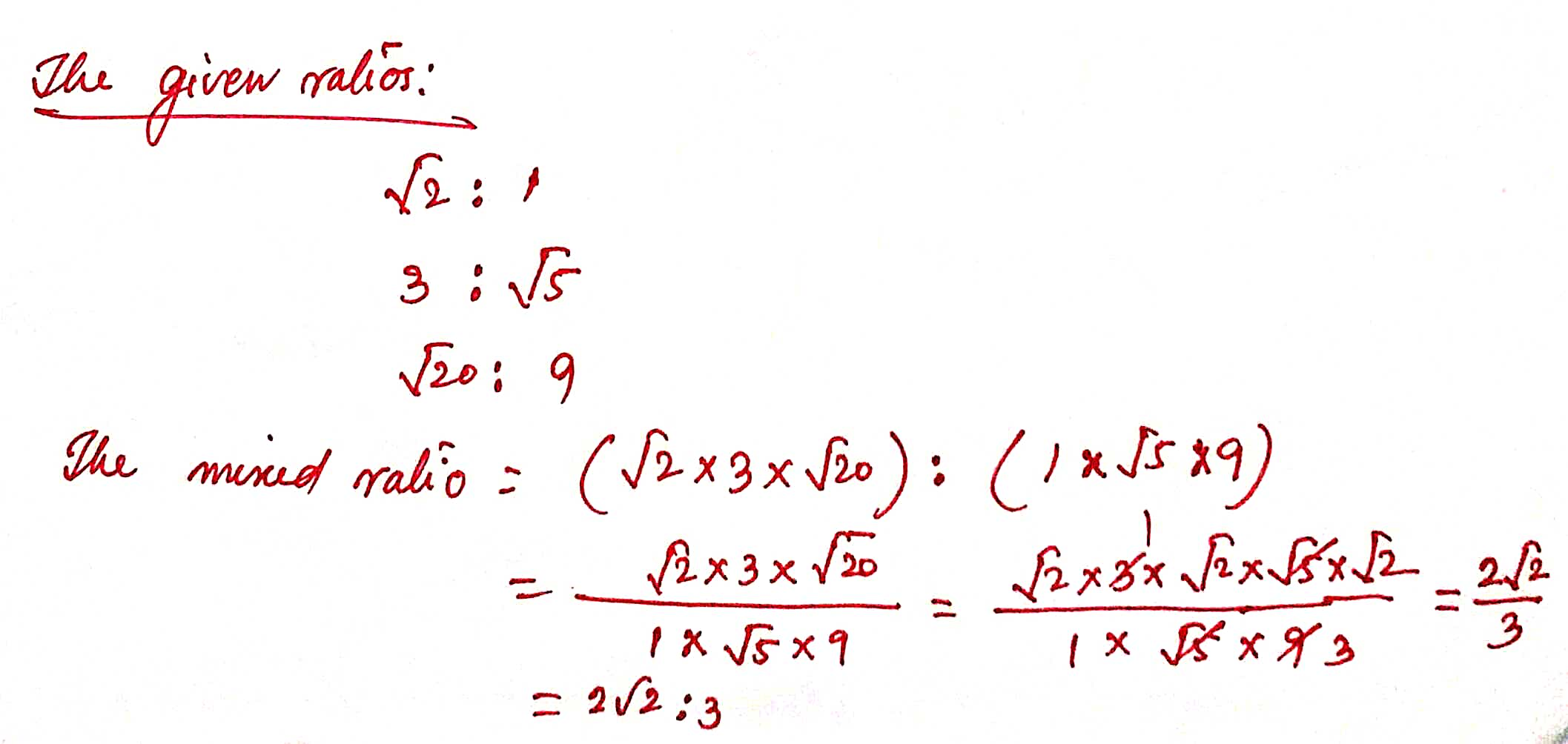18 ) Find the compound ratio of the inverse ratio of 1 : 3, 3 : 4 and 64 : 27.

Solution :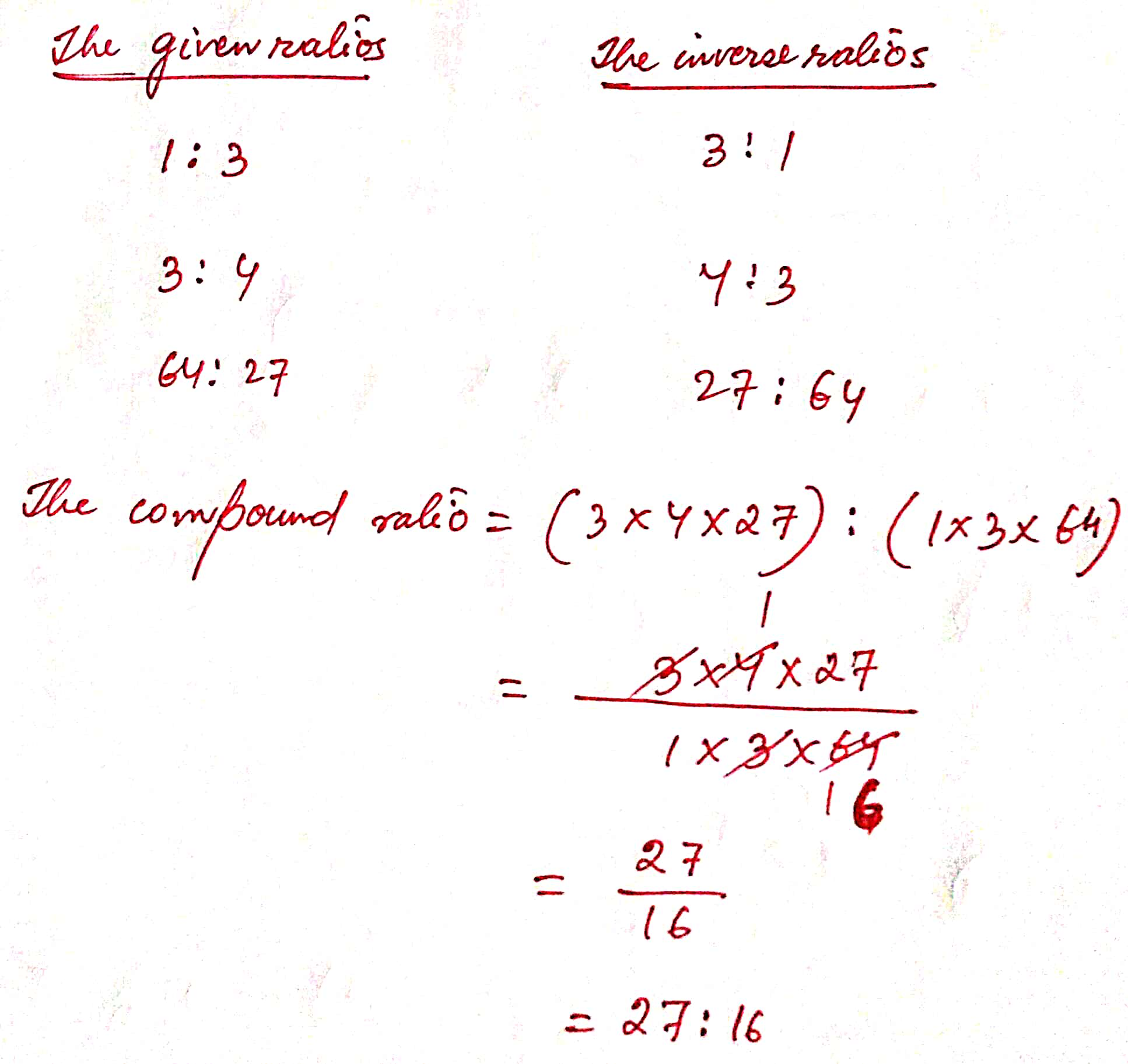19 ) If x : y = 4 : 5 and y : z = 6 : 7 then find x : z.

Solution :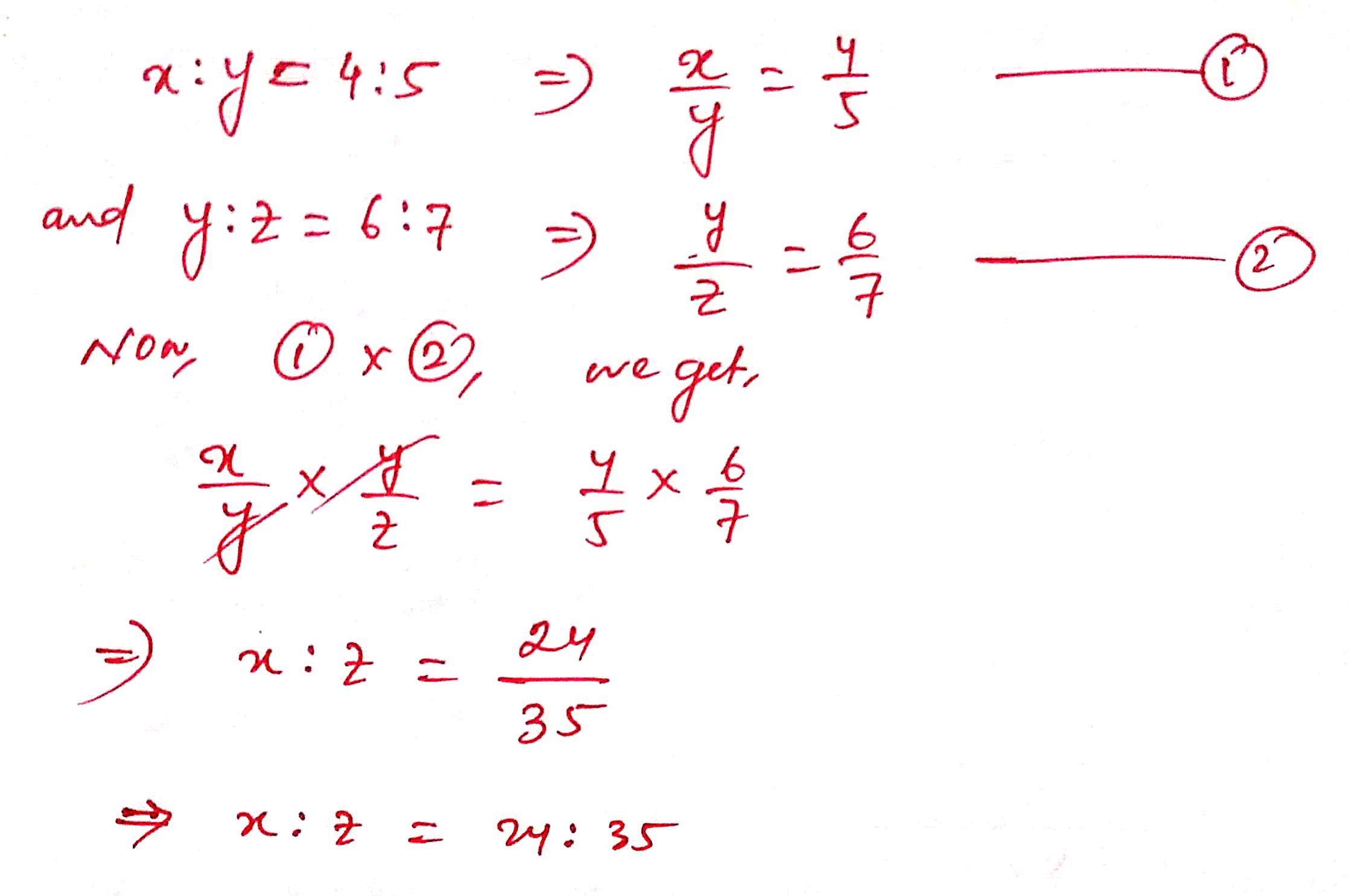20 ) If A : B = 3 : 7 and B : C = 8 : 5 then calculate A : C.

Solution :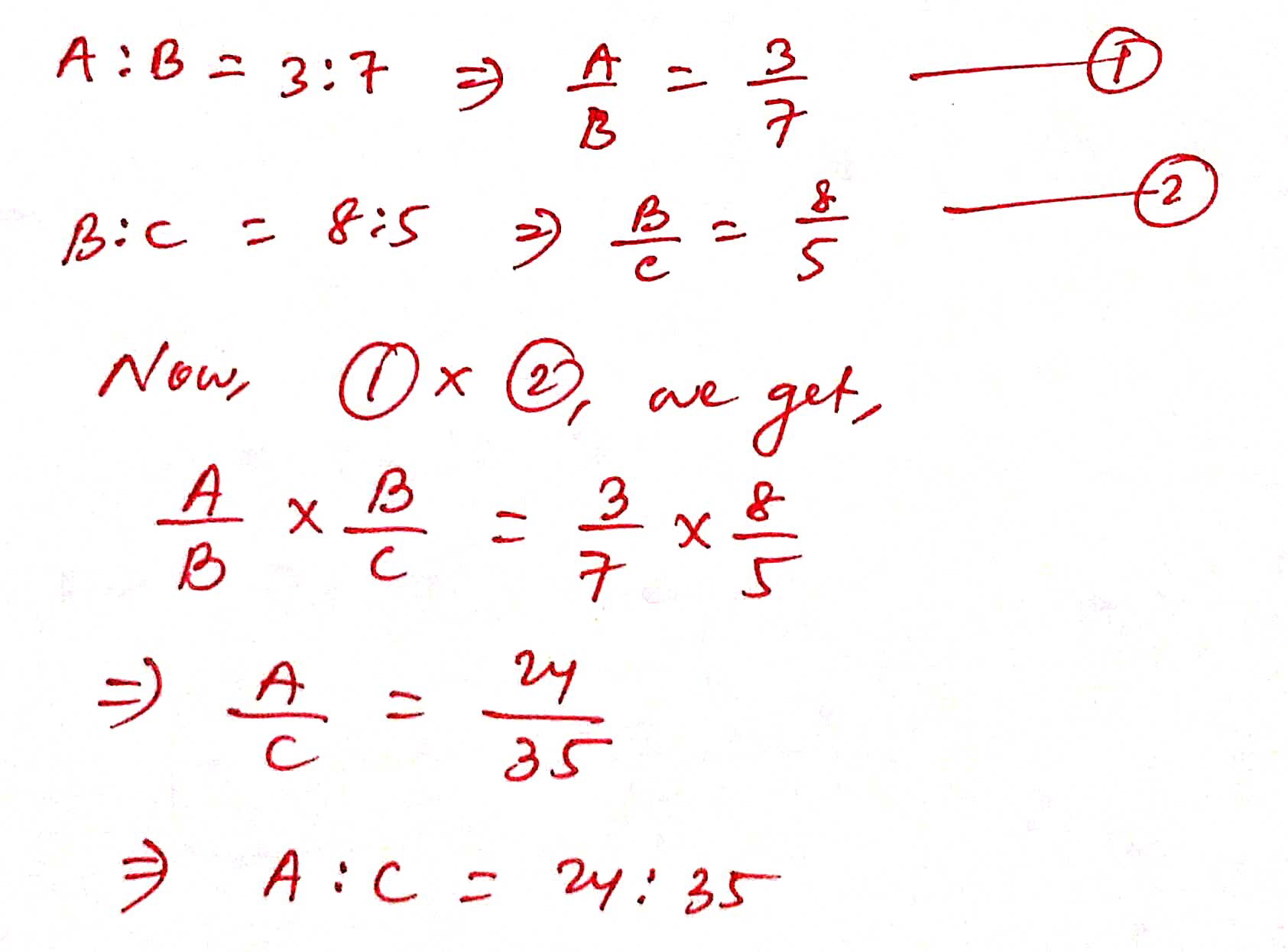21 ) If a : b = 2 : 3, b : c = 4 : 5 and c : d = 6 : 7 find a : d

Solution :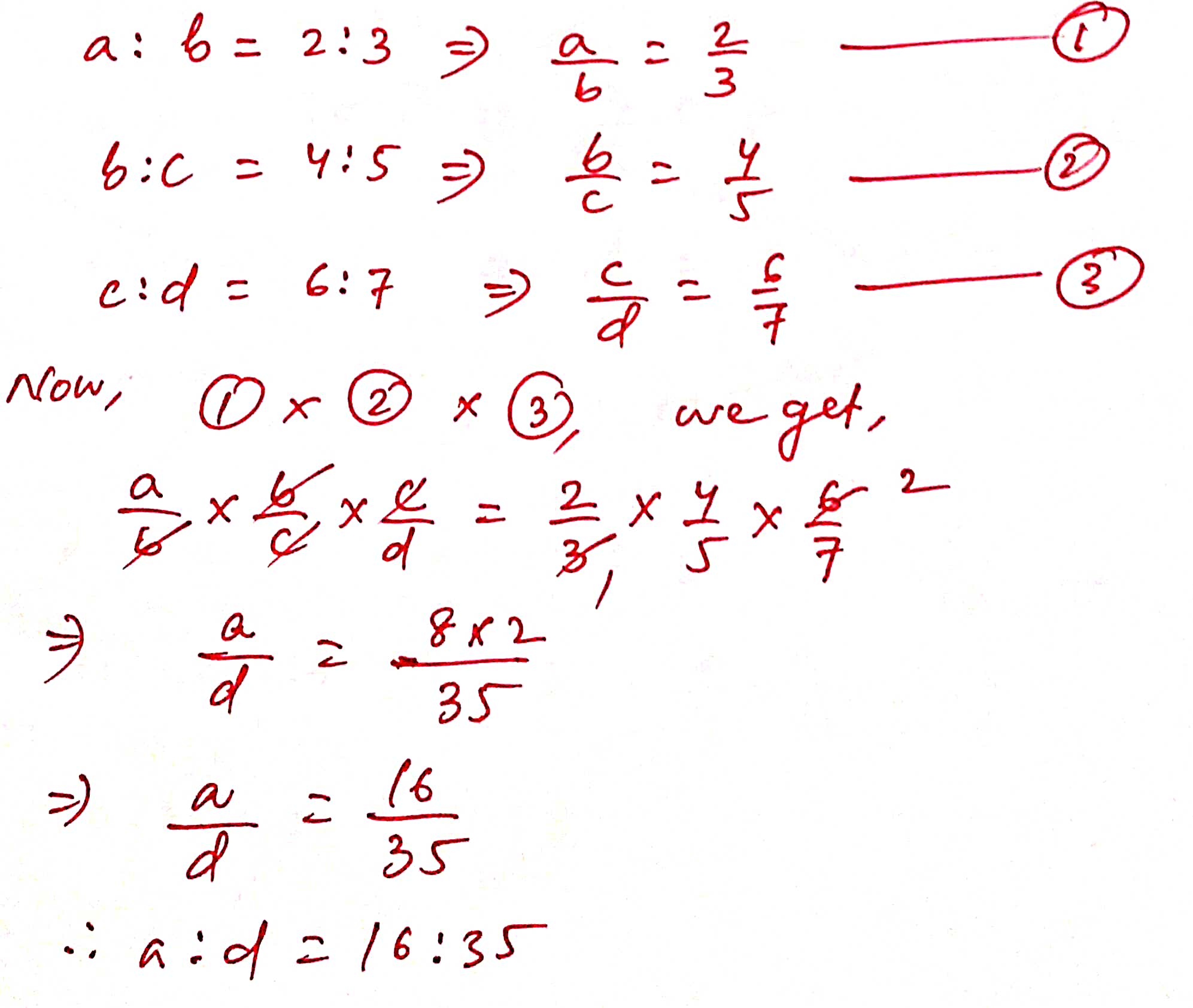22 ) If a : b = 6 : 7 and b : c = 8 : 7 find a : c

Solution :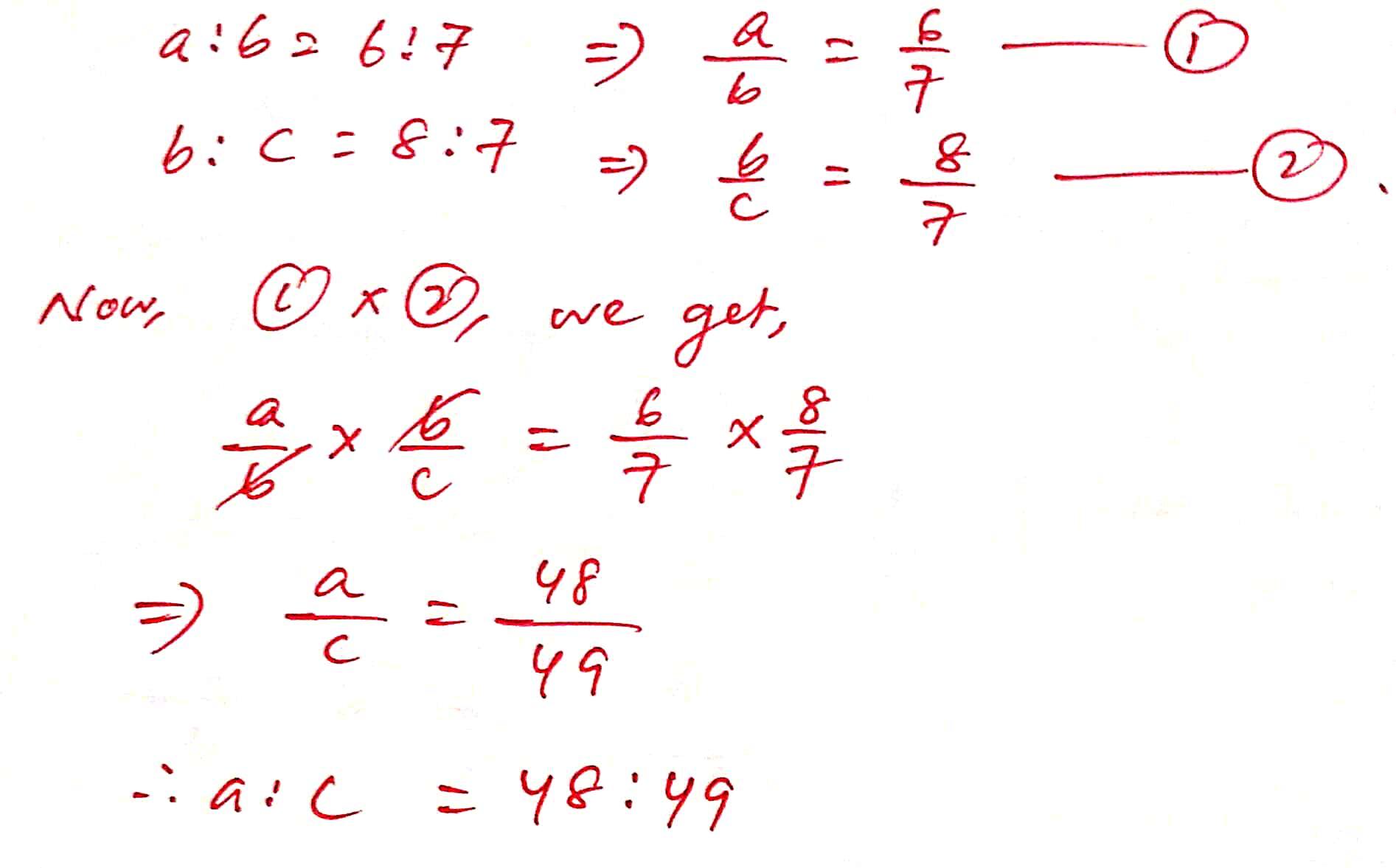23 ) If a : b = 3 : 4 and b : c = 6 : 7 then find out a : b : c and a : c

Solution :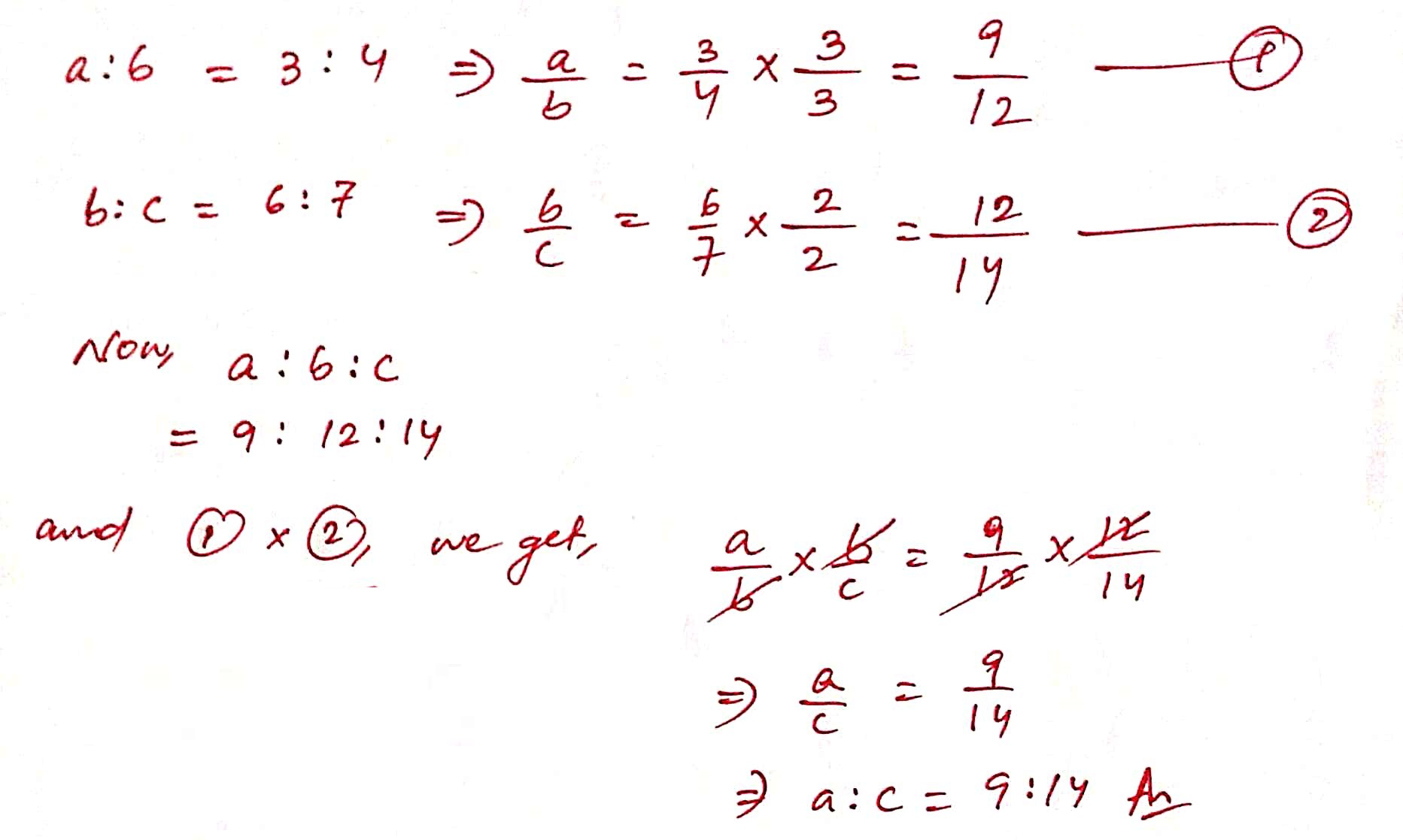24 ) If a : b = 2 : 5 and a : c = 3 : 4 then find a : b : c.

Solution :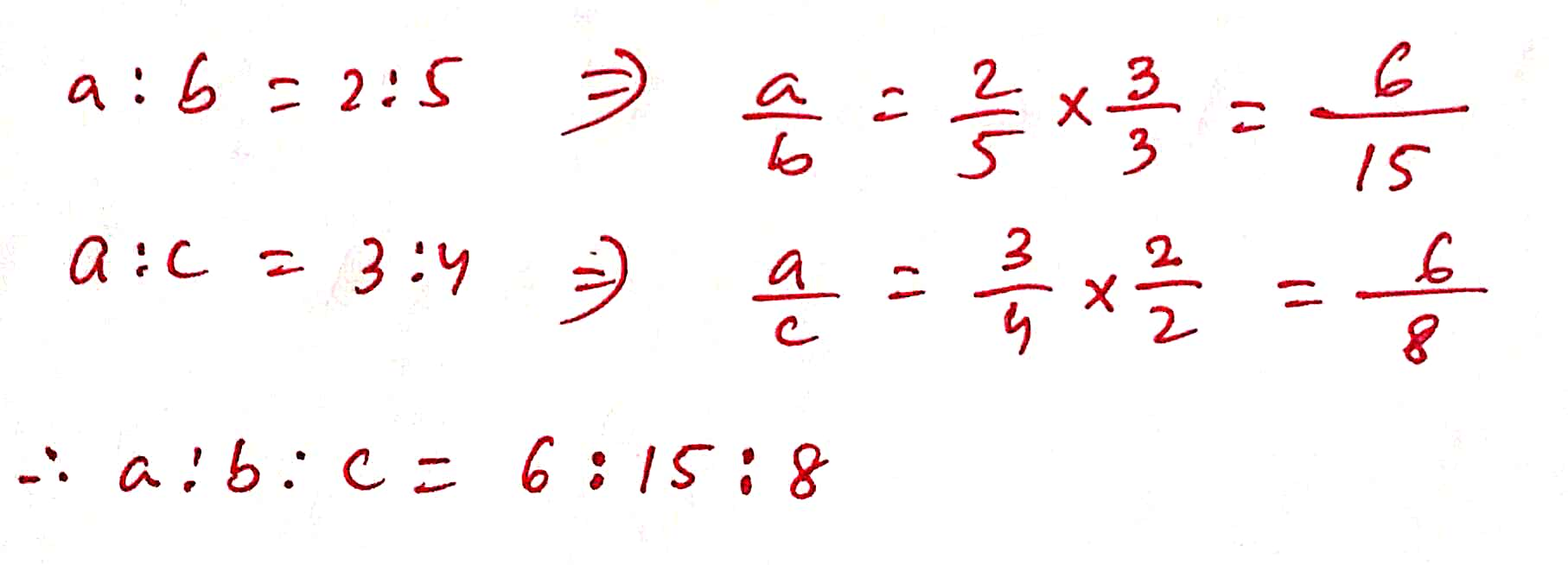25 ) If 3x = 4y = 6z , find x : y : z

Solution :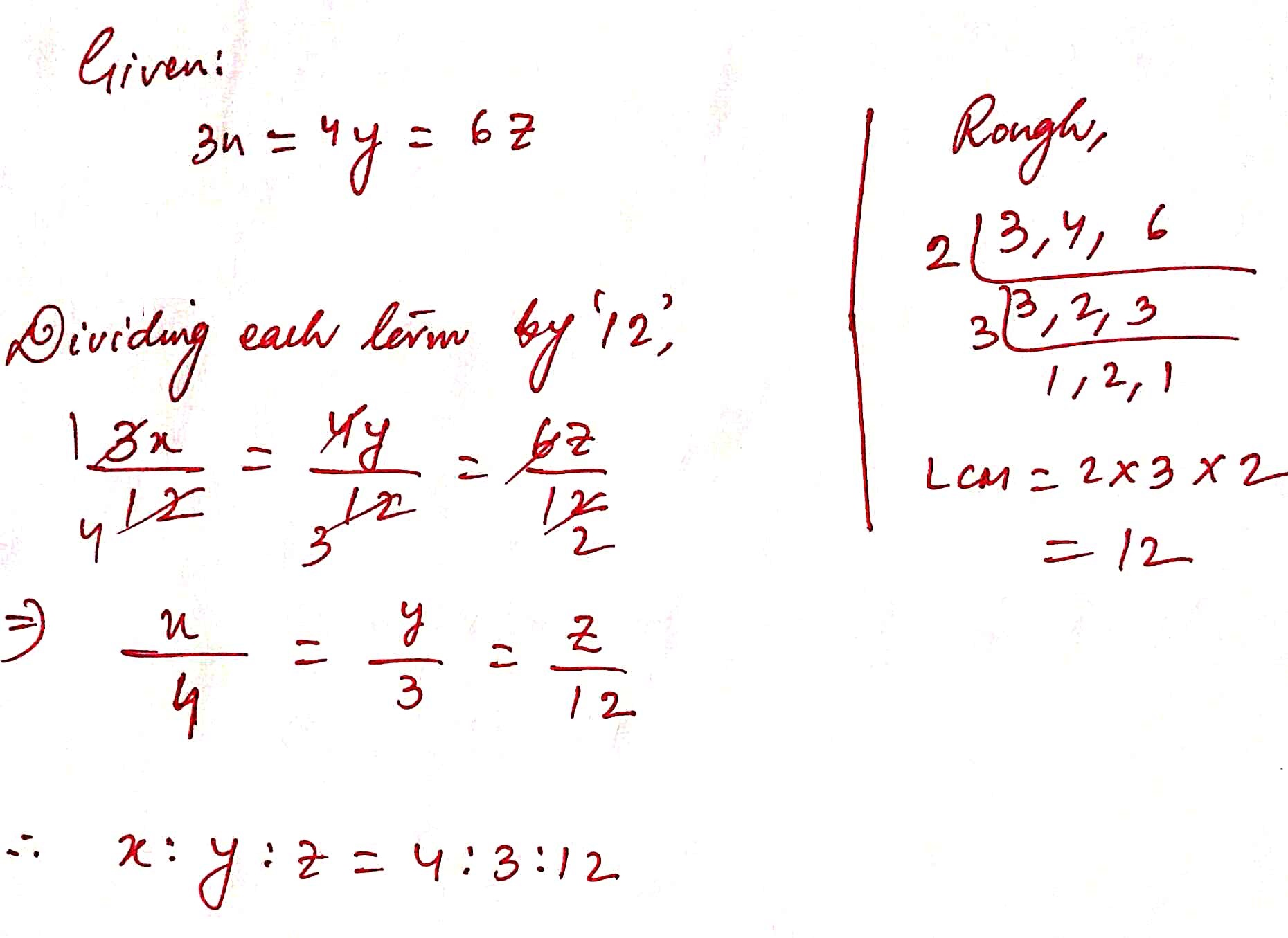26 ) If 2a = 3b and 4b = 5c , find a : c

Solution :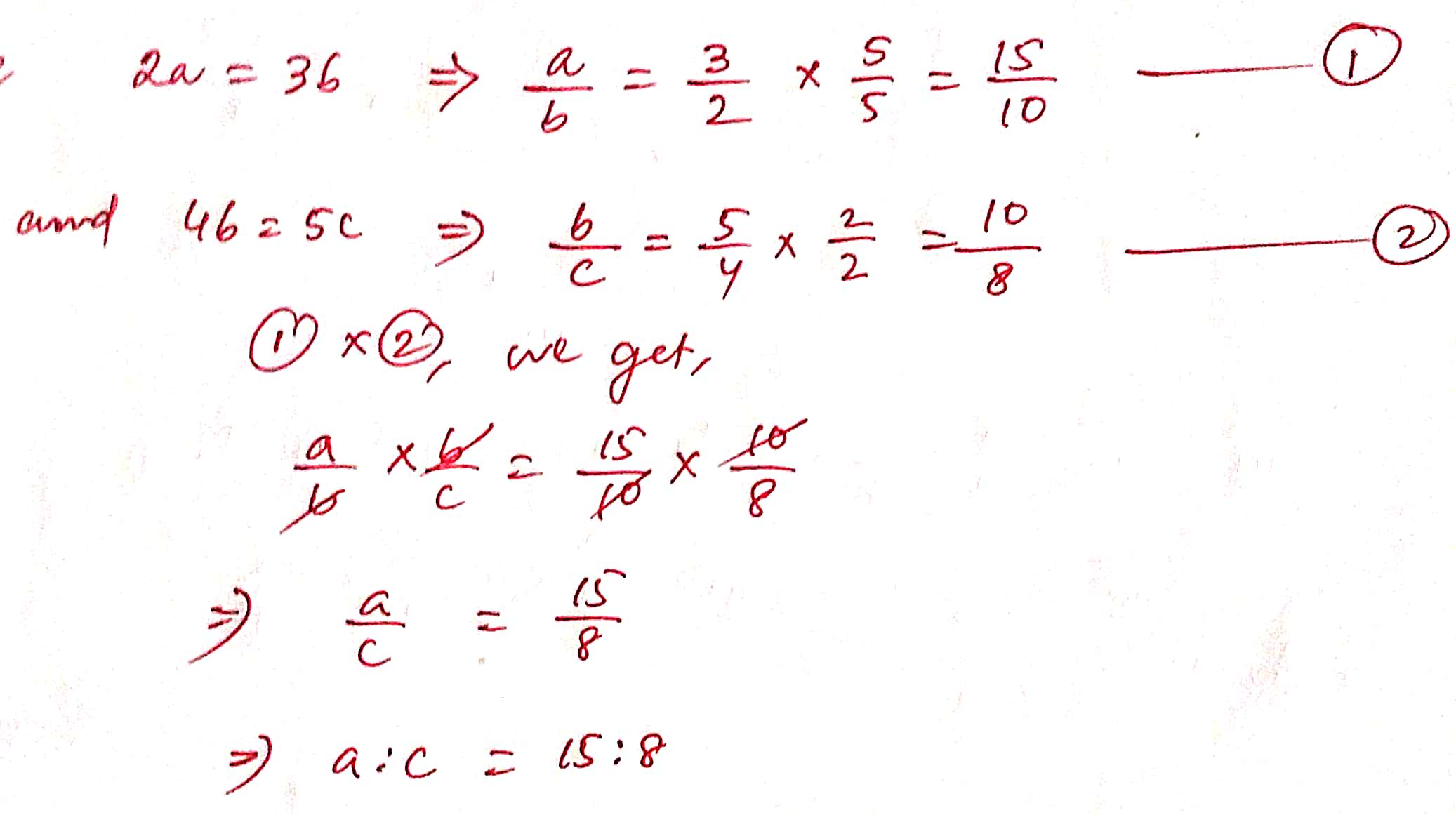27 ) If x : y = 2 : 3 and y : z = 4 : 7, find x : y : z

Solution :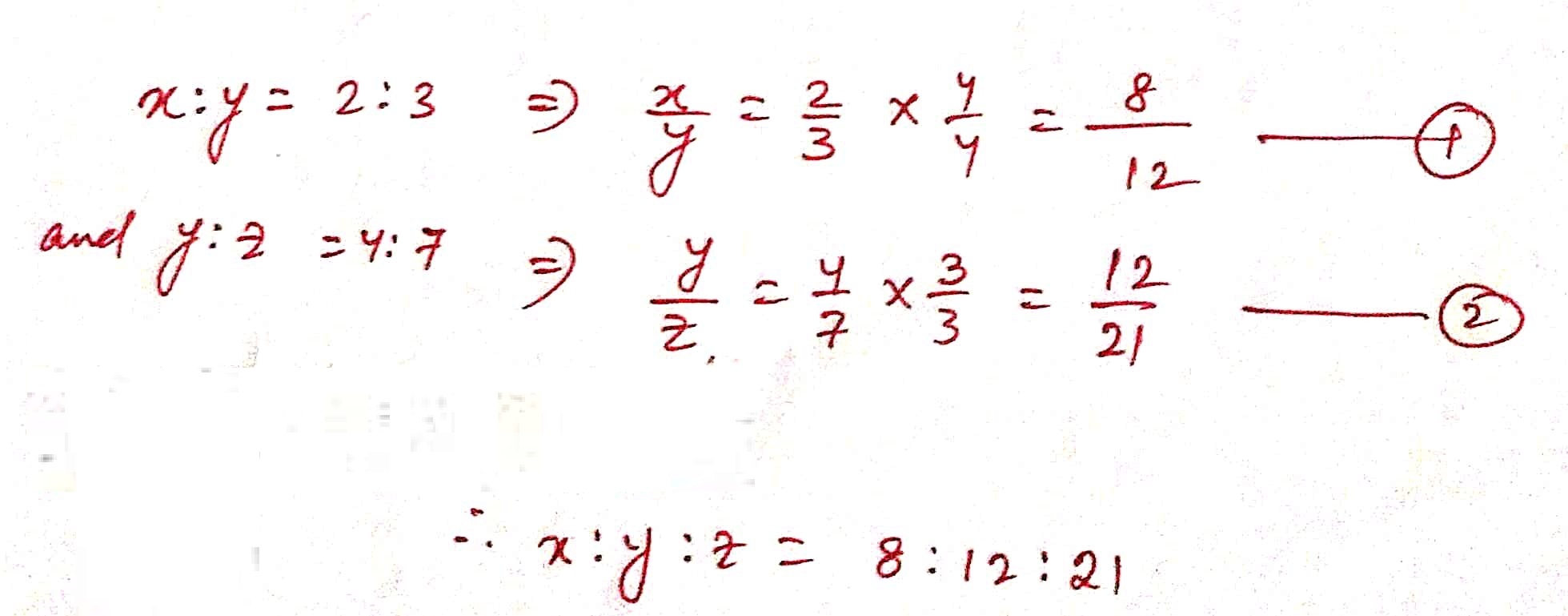28 ) If p : q = 5 : 7 and p – q = – 4 , find the value of 3p + 4q.

Solution :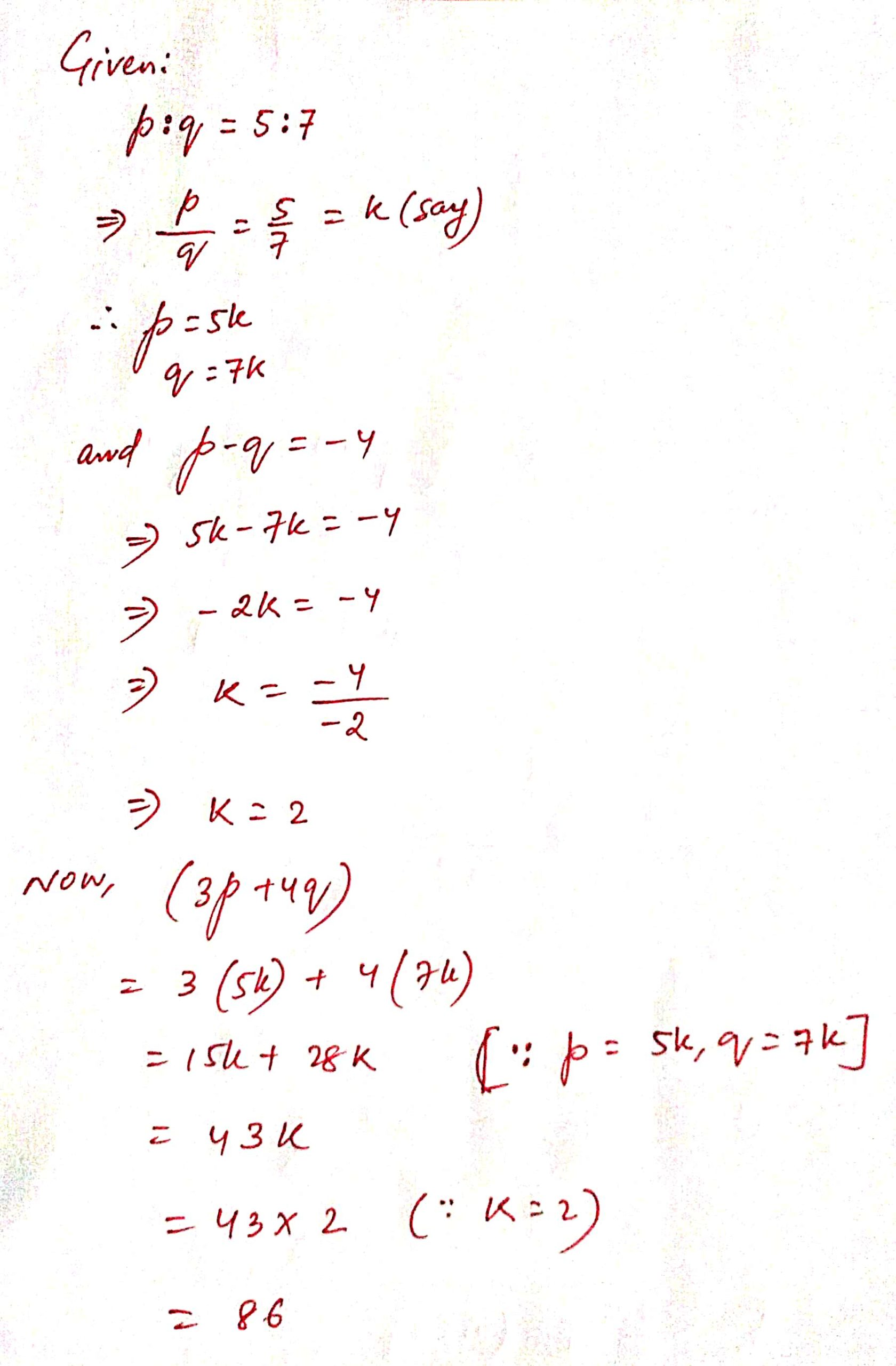29 ) If a : b = 5 : 3 , find ( 5a -3b ) / ( 5a + 3b )

Solution :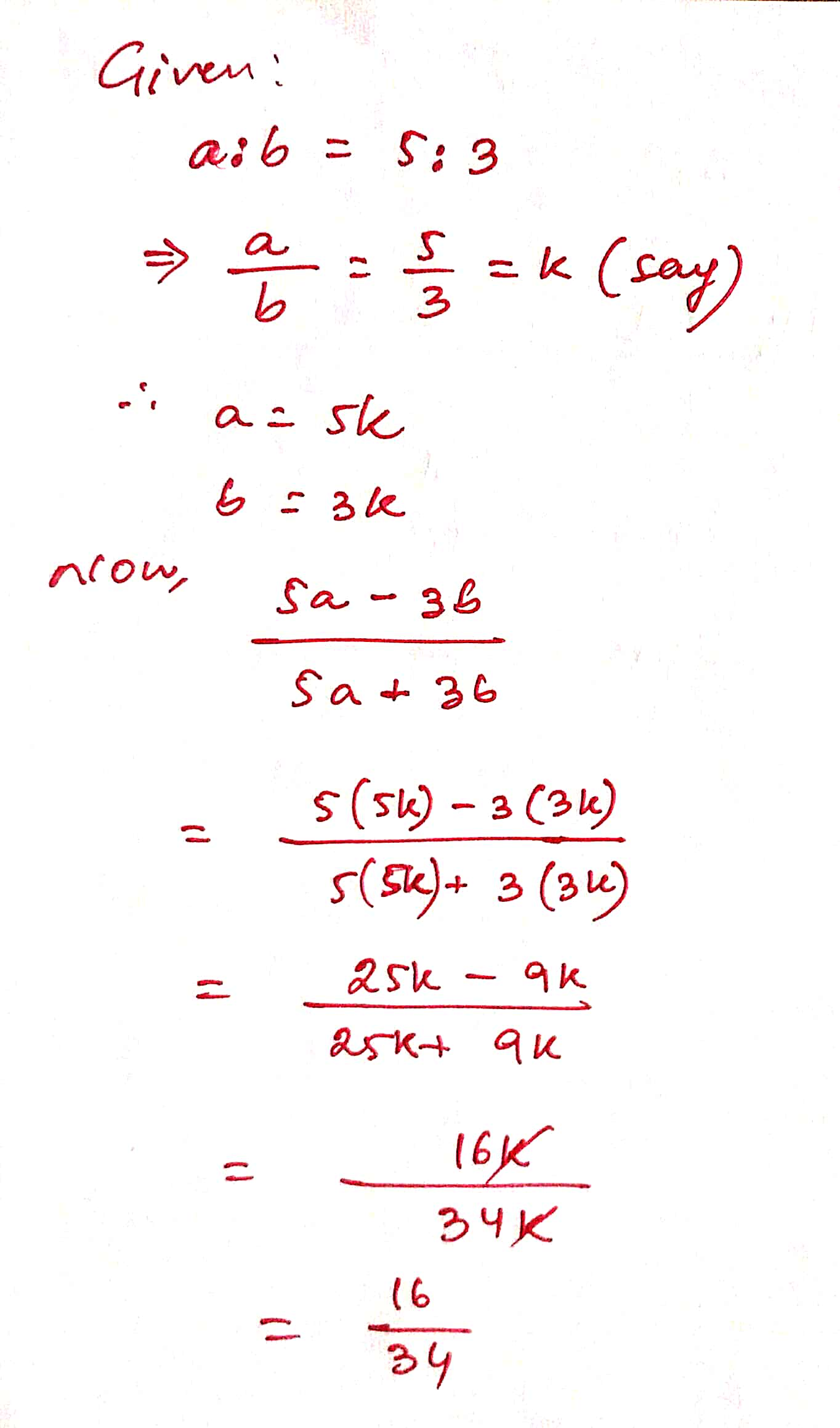30 ) If x : y = 4 : 7 find the value of ( 3x + 2y ) : ( 5x + y ).

Solution :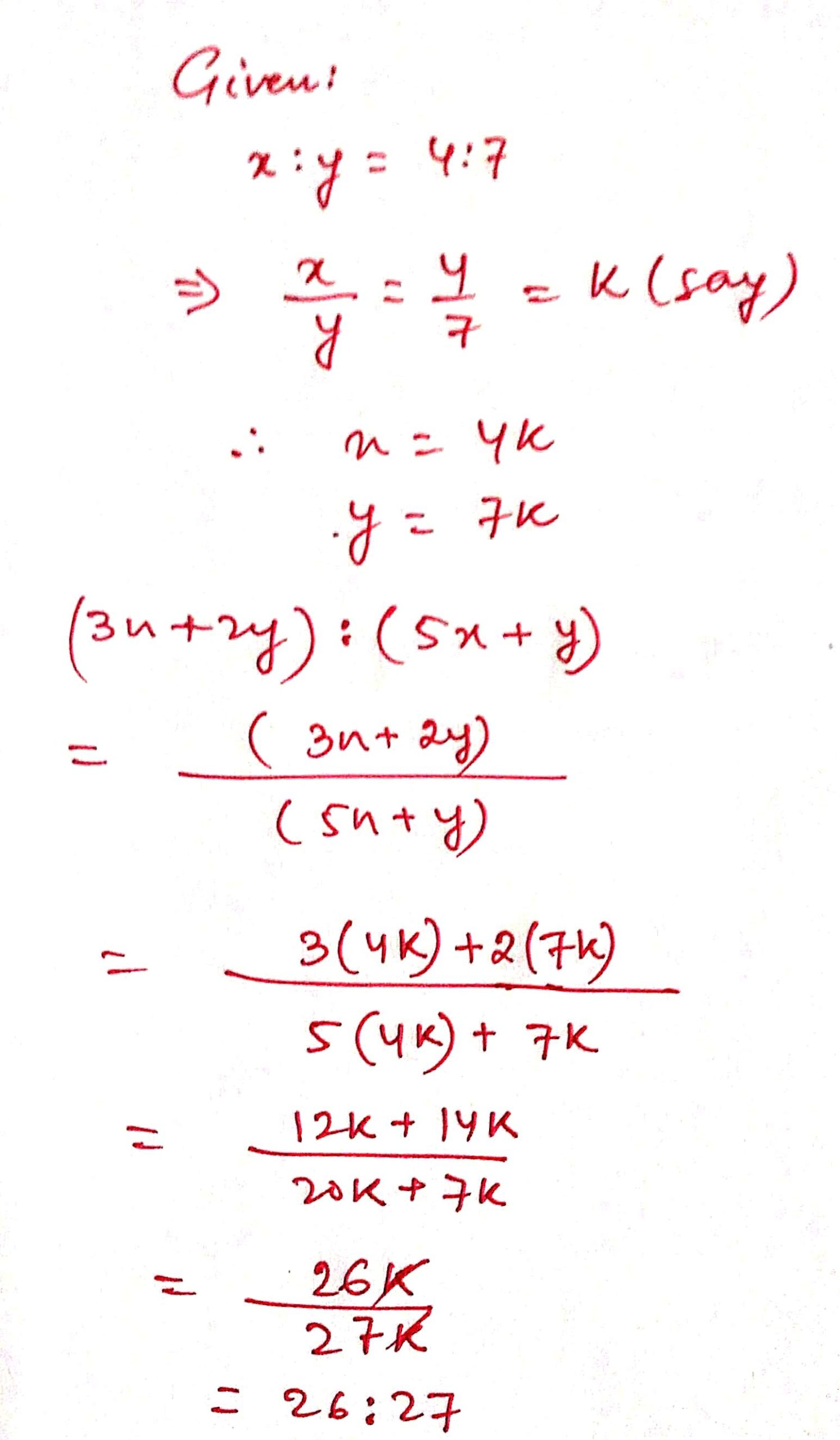31 ) If ( a – b ) : ( a + b ) = 1: 11 find the value of ( 5a + 4b) : ( 5a – 4b )

Solution :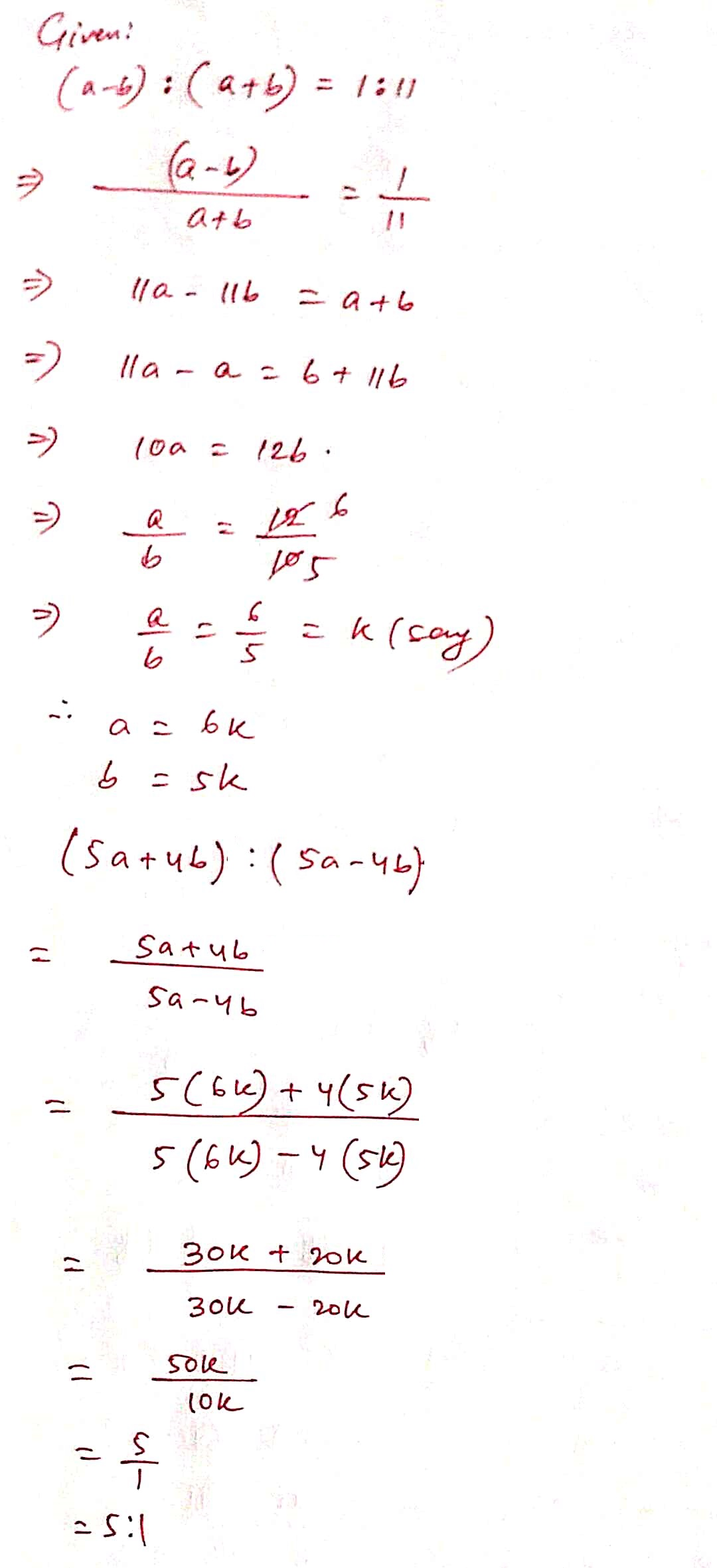32 ) If ( m + n ) / ( m + 3n ) = 2/3, find 2n / ( 3m + mn ).

Solution :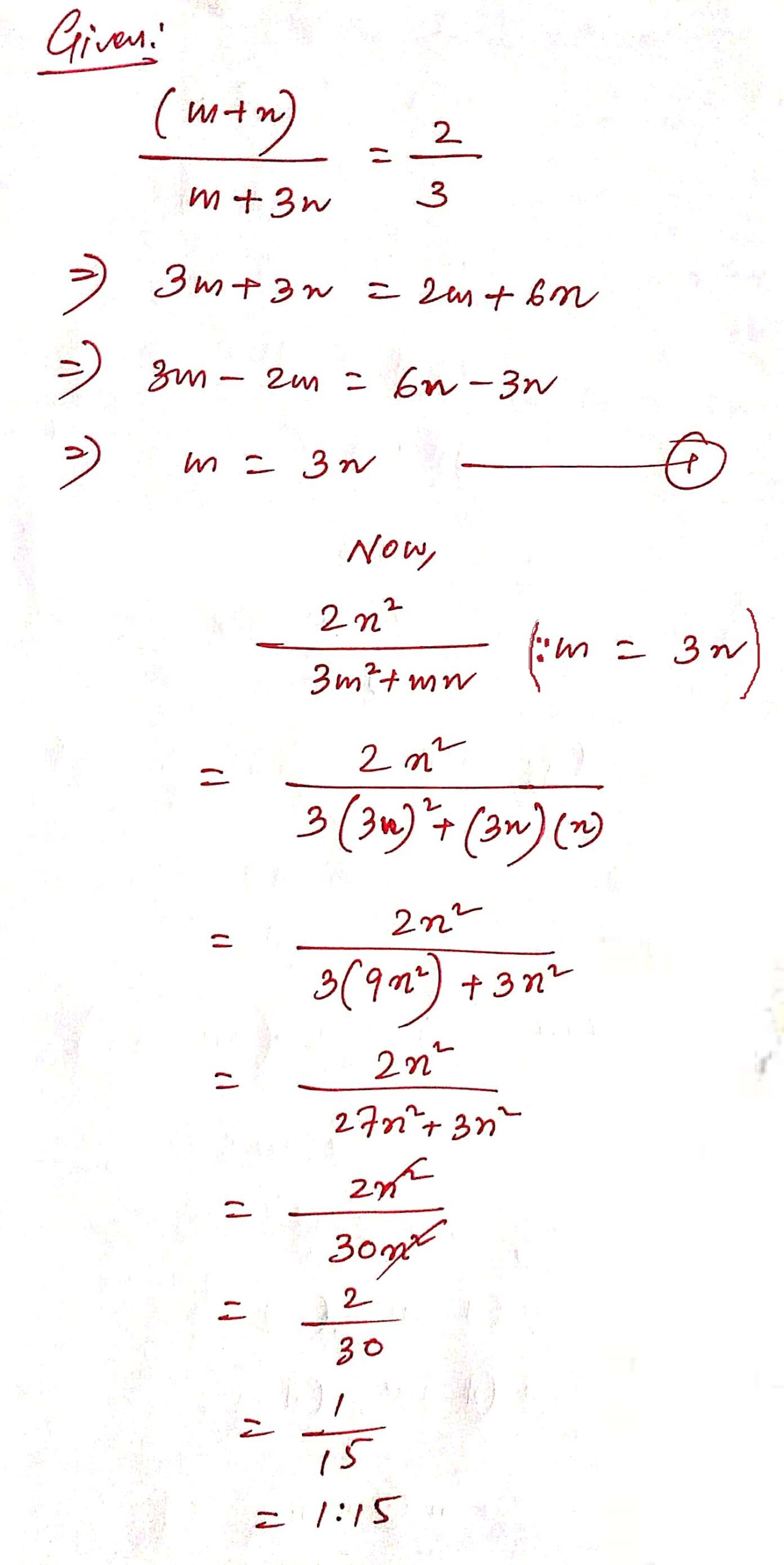33 ) Find x /y when x + 6y = 5xy.

Solution :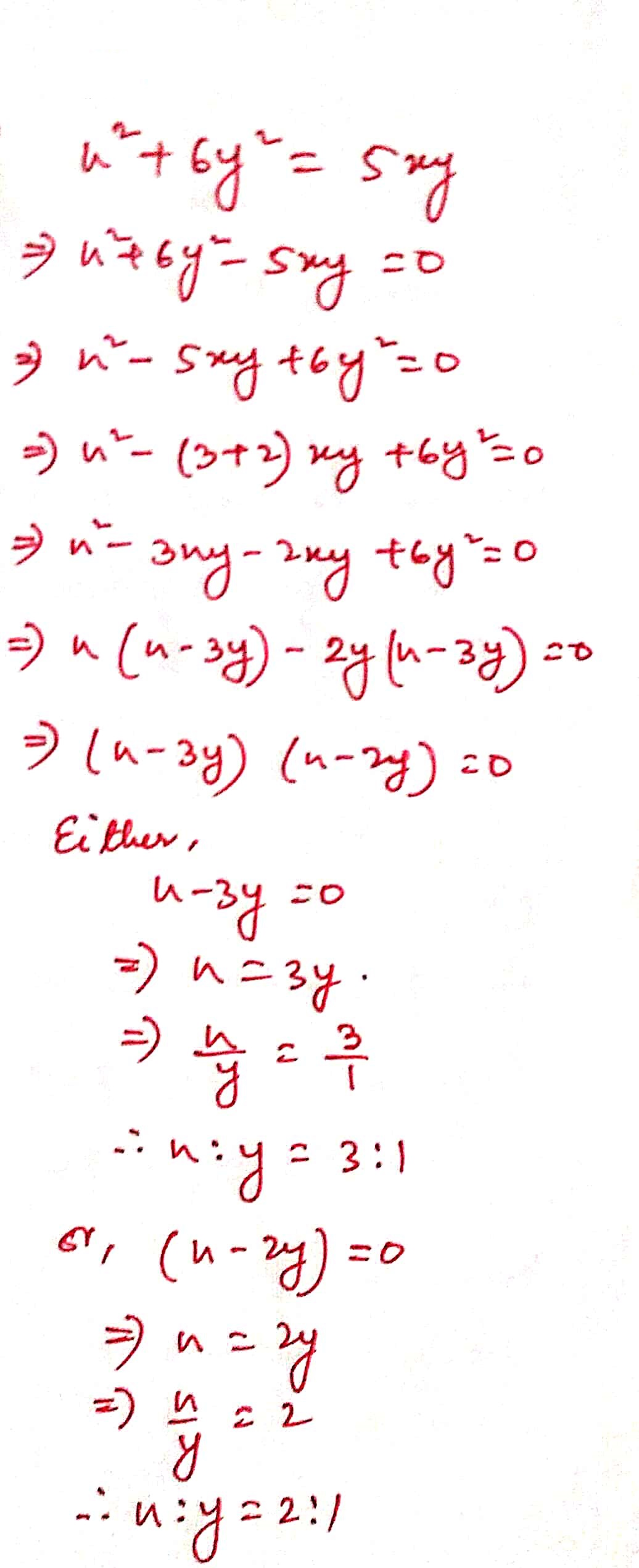34 ) If a : b = 5 : 3 find ( 5a + 8b ) : ( 6a – 7b )

Solution :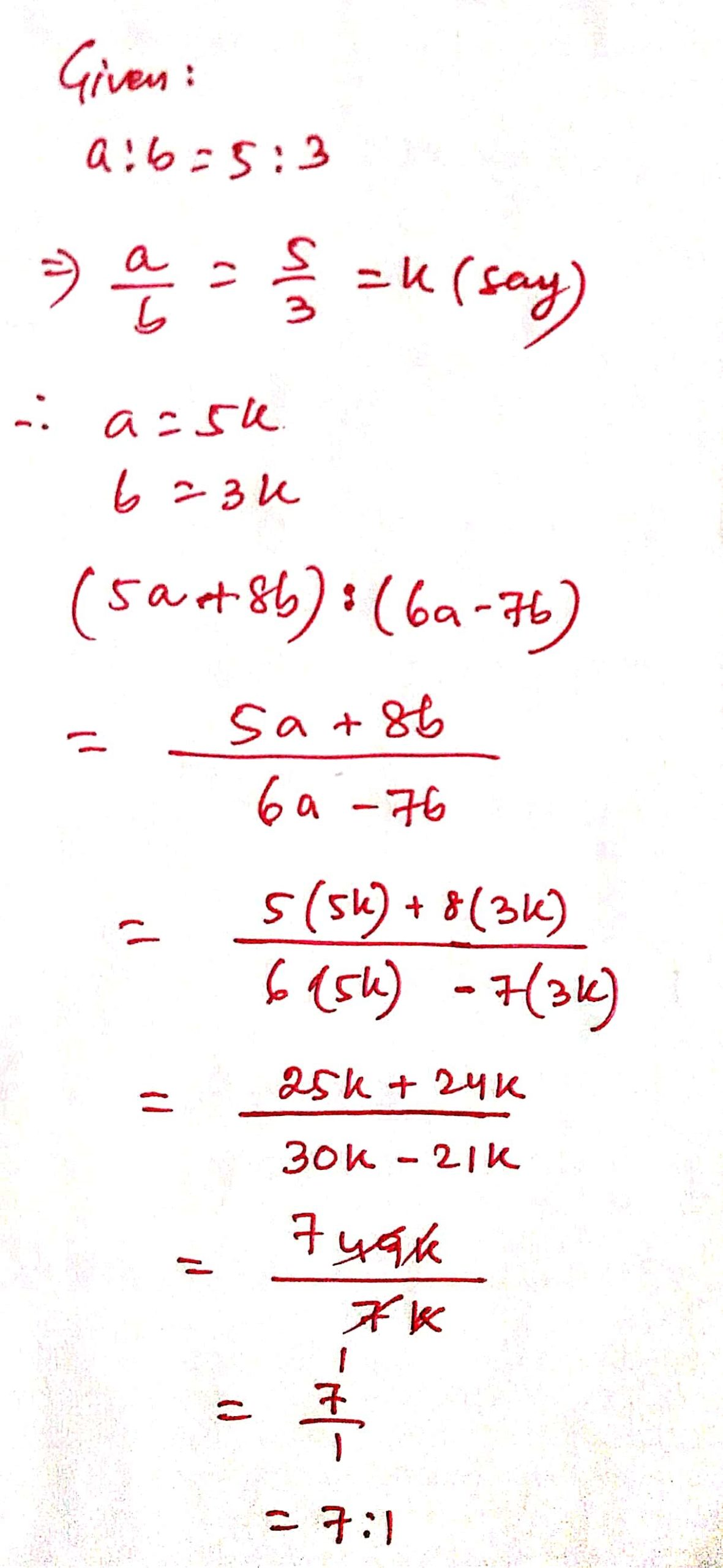35 ) If x : y = 7 : 4 show that ( 5x – 6y ) : ( 3x + 11y ) = 11 : 65

Solution :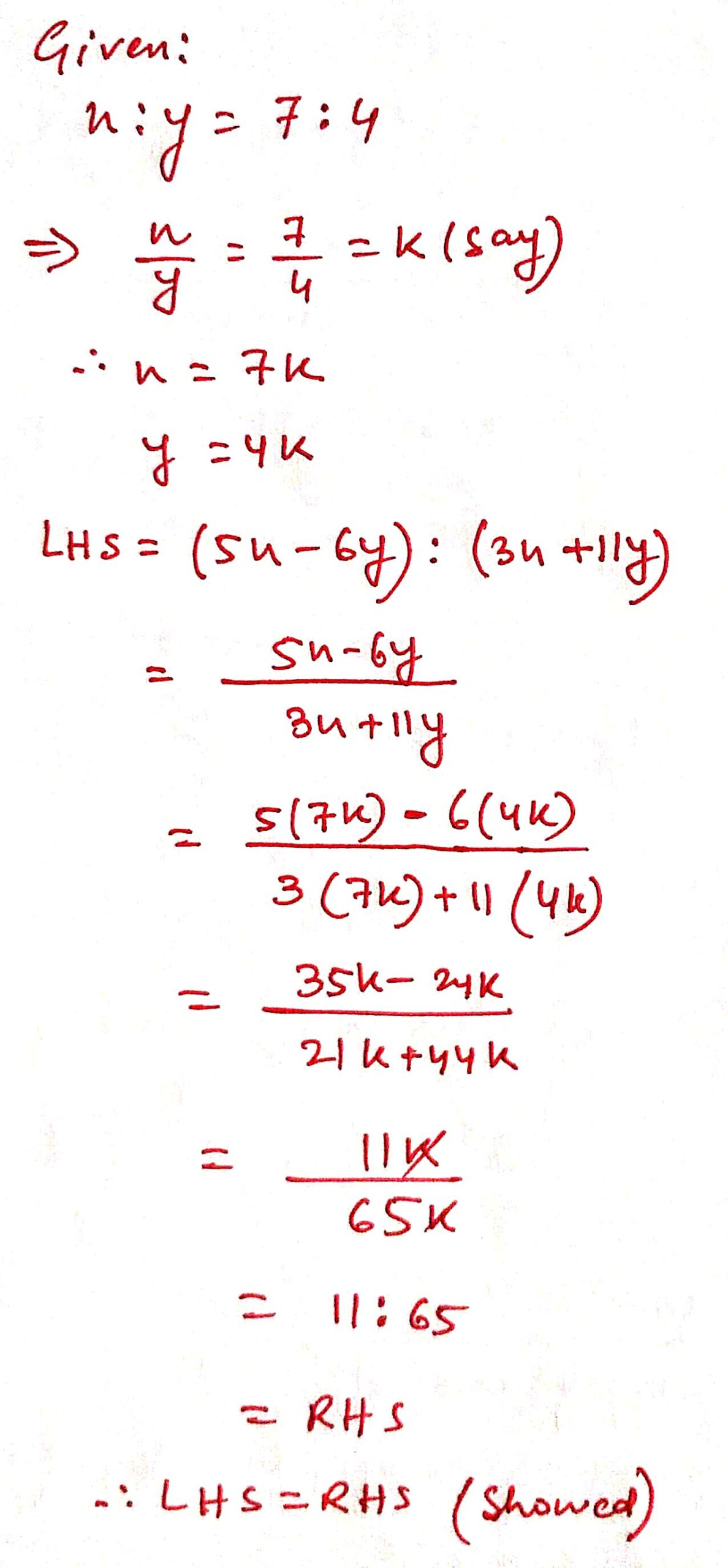36 ) If ( 2x + 5y ) : ( 5x – 7y ) = 5 : 3 find x : y

Solution :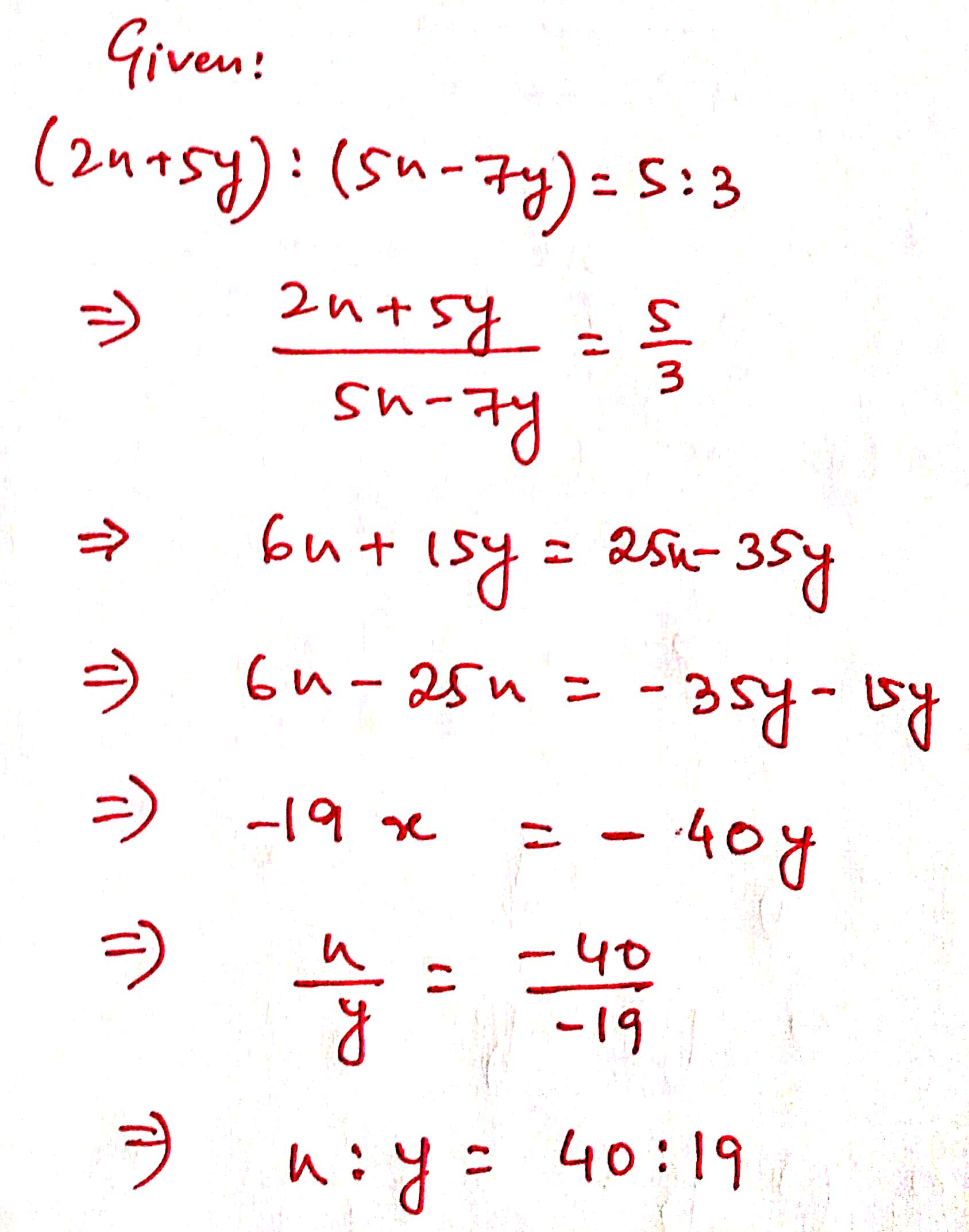37 ) If ( 7x – 5y ) : ( 3x + 4y ) = 7 : 11 find out the value of ( 5x -3y ) : ( 6x + 5y ).

Solution :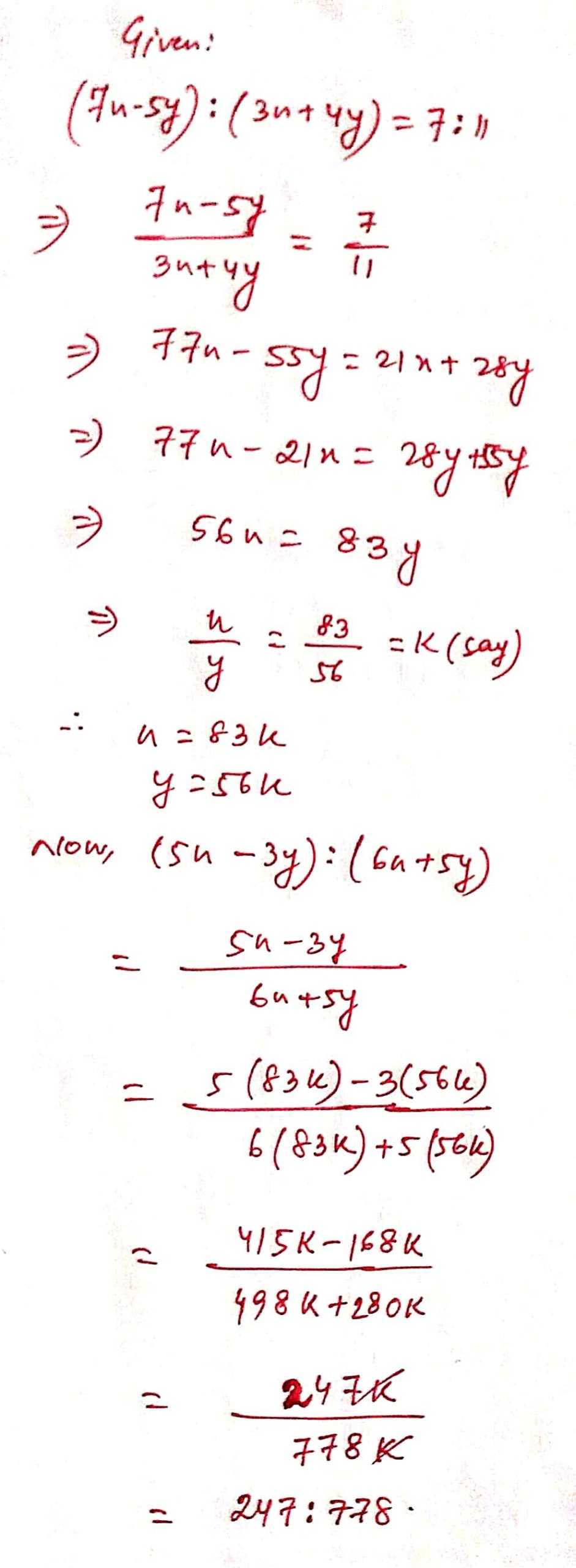38 ) What should be added to each term of the ratio 5 : 3 to make the ratio 7 : 6

Solution :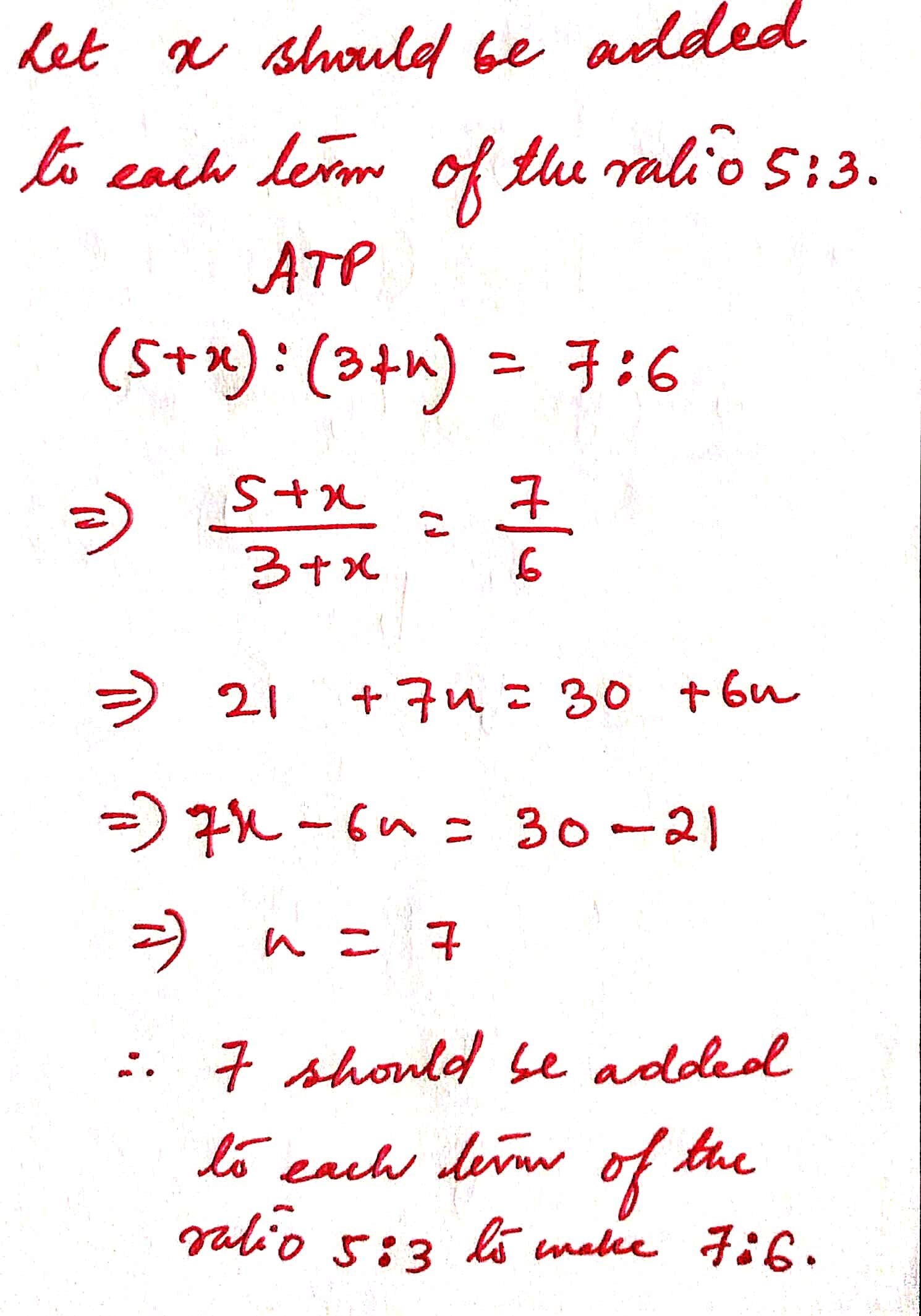39 ) If ( 7x – 5y ) : ( 3x + 4y ) = 7 : 11, prove that ( 3x – 2y ) : ( 3x + 4y ) = 137 : 473.

Solution :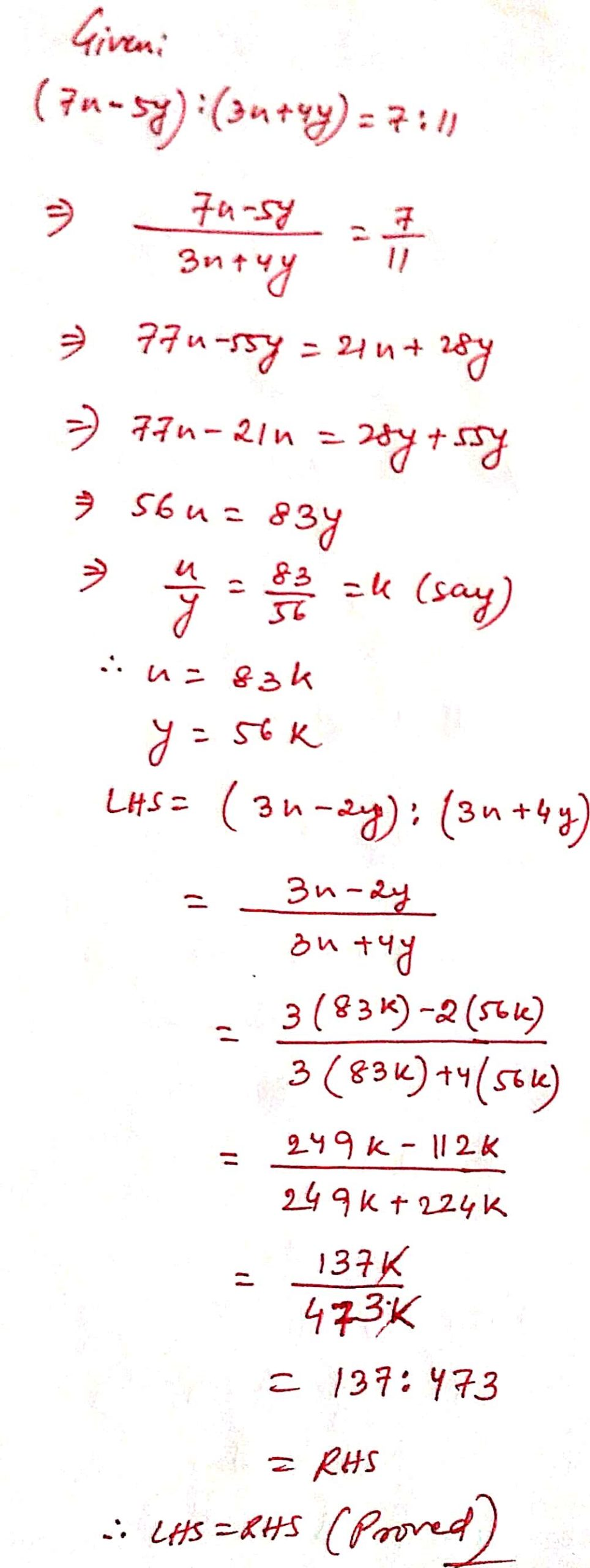40 ) If ( 10 x + 3y ) : ( 5x + 2y ) = 9 : 5 then show that ( 2x + y ) : ( x + 2y ) = 11 : 13 .

Solution :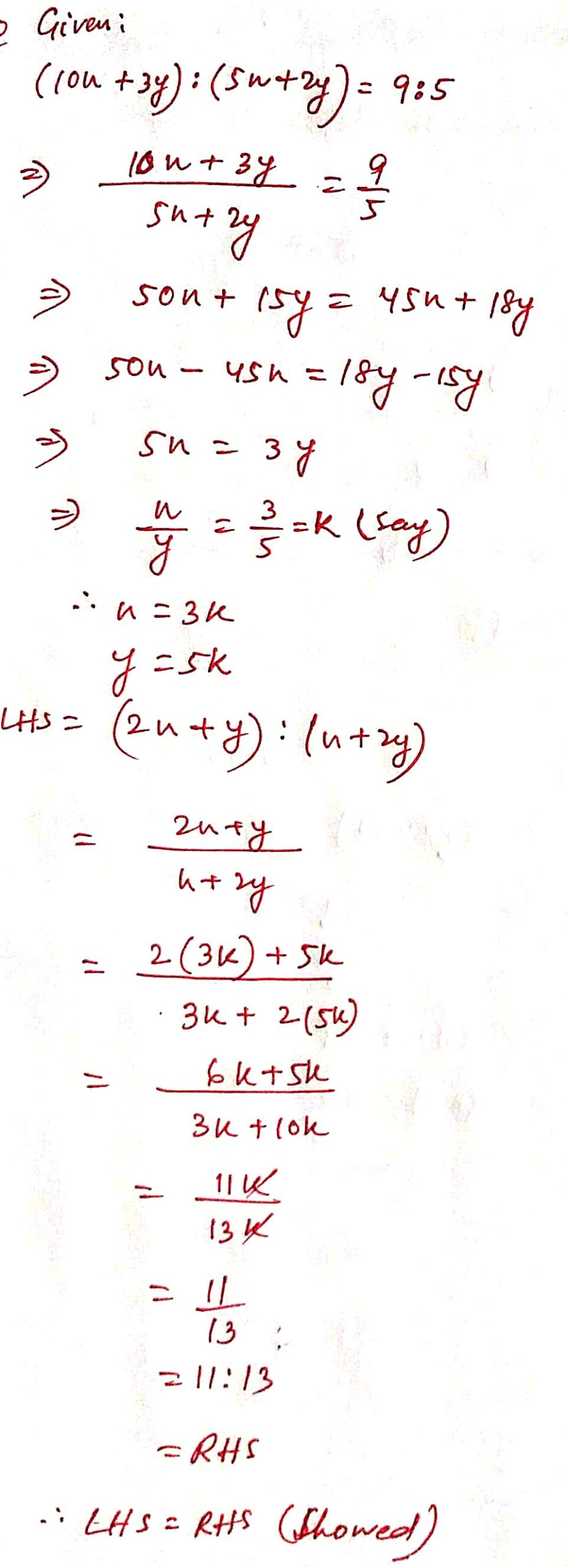41 ) Calculate what term should be added to both terms of the ratio 2 : 5 to make the ratio 6 : 11.

Solution :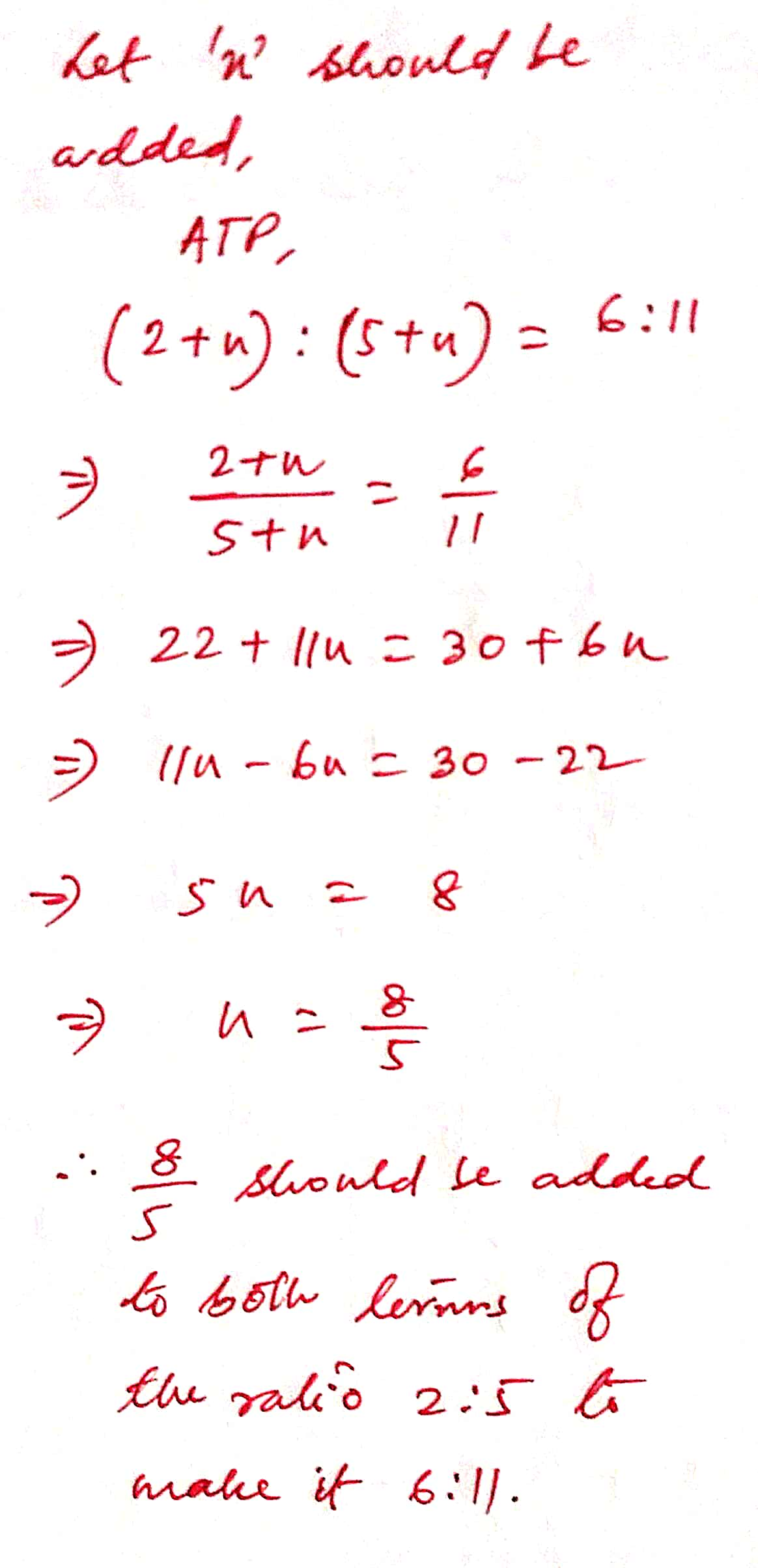42 ) What term should be subtracted from each term of the ratio a : b to make the ratio m : n ?

Solution :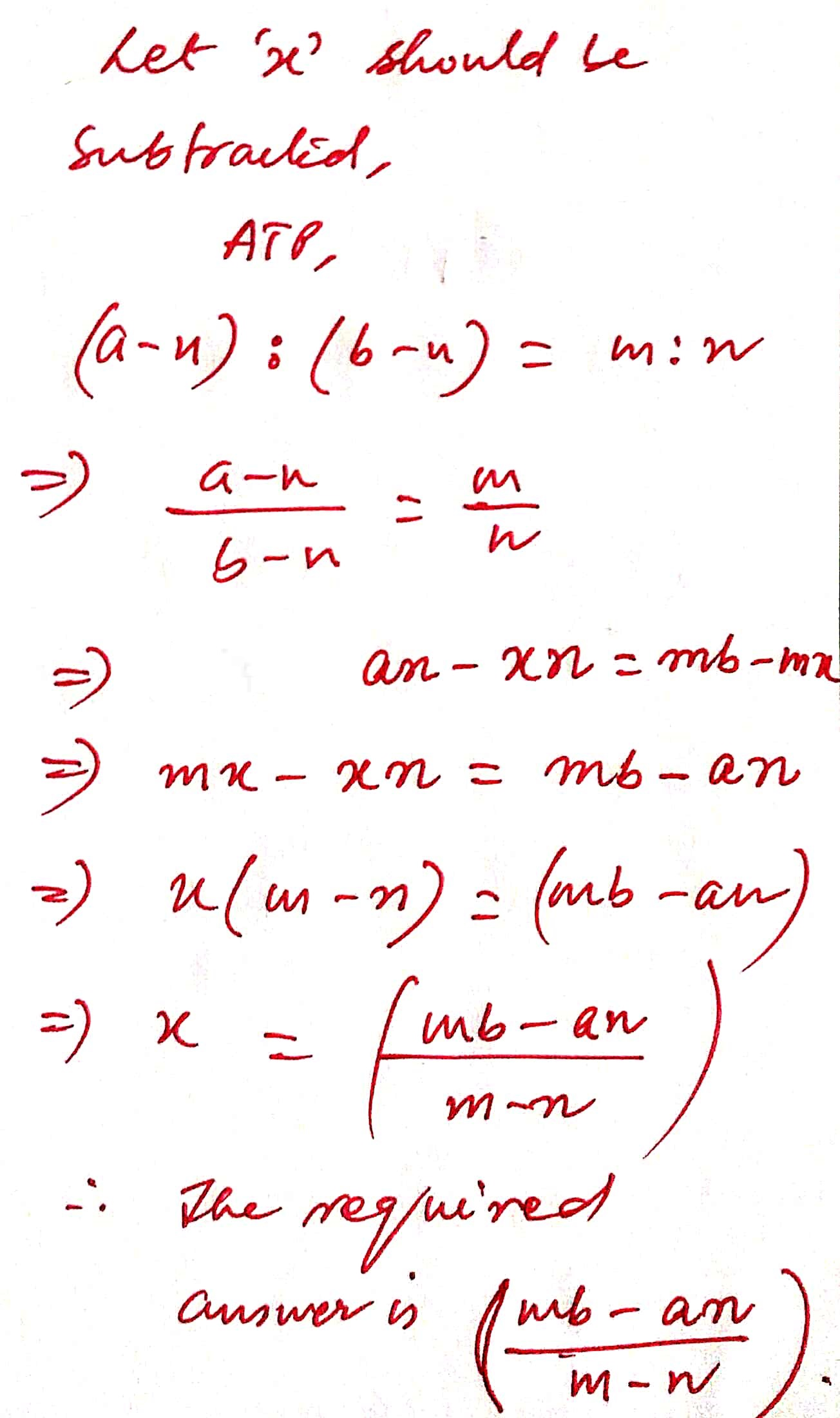43 ) What term should be added to antecedent and subtracted from consequent of ratio 4 : 7 to make a compound ratio of 2 : 3 and 5 : 4 ?

Solution :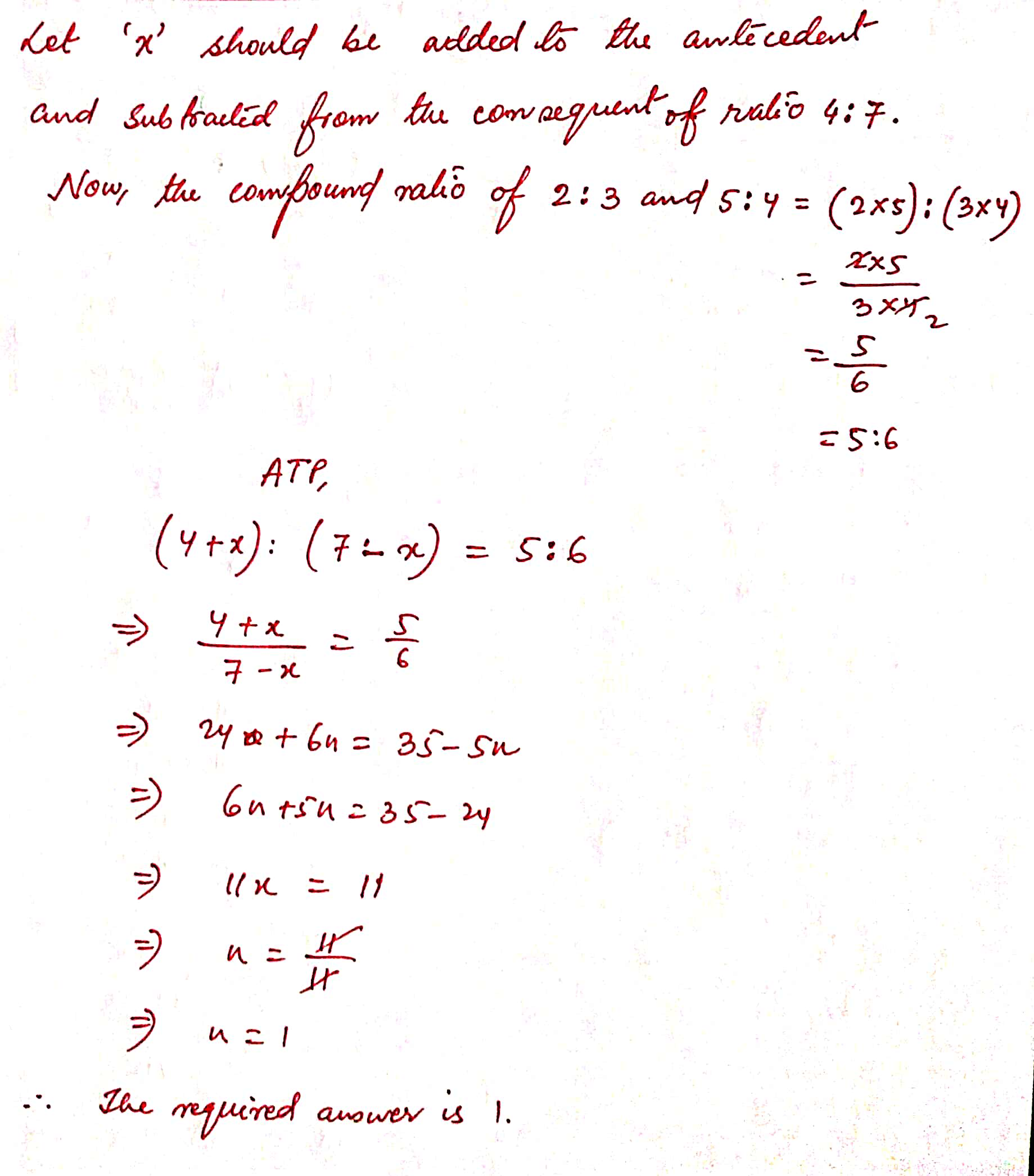44 ) What term should be subtracted from each term of the ratio x : y to make the ratio p : q ?

Solution :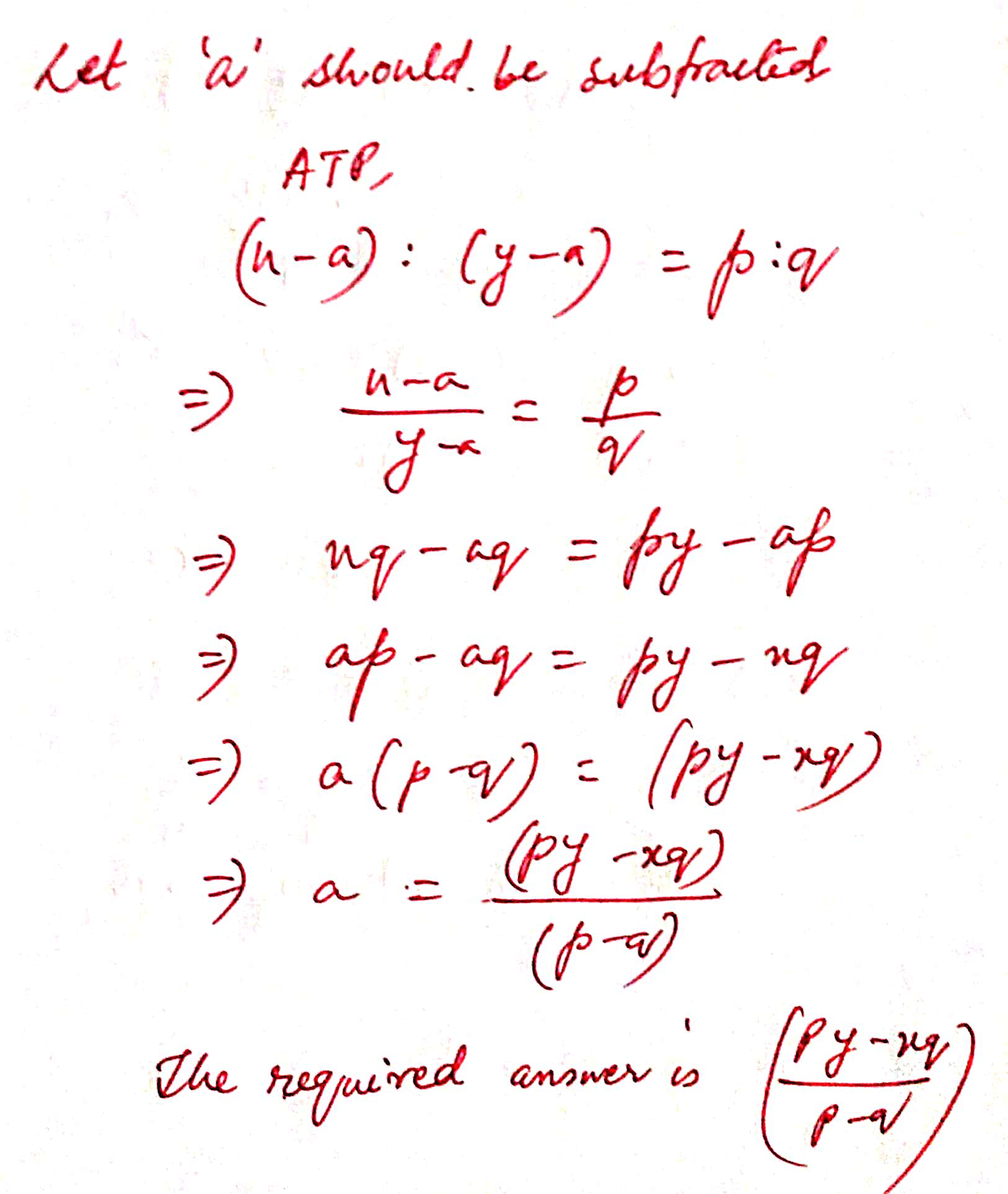45 ) Find the inverse ratio of the mixed ratio of three ratios p : q , q2 r : p and r p : q.

Solution :